Martin Flashman's Courses
MATH 316 Real Analysis I Spring, 2016
Class Notes and Summaries
[Based on notes from 2013]
 Monday Tuesday Thursday Friday Week1: Beginnings. 1-18 MLK day 1-19 1-21 1-22 Week 2 1-25 1-26 1-28 1-29 Week 3 2-1 2-2 2-4 2-5 Week 4 2-8 2-9 2-11 2-12 Week 5 2-15 2-16 2-18 2-19 Week 6 2-22 2-23 2-25 2-26 Week 7 2-29 3-1 3-3 3-4 Week 8 3-7 3-8 3-10 3-11 Week 9 Spring Break Week 10 3-21 3-22 3-24 3-25 Week 11 3-28 3-29 3-31 CC Day 4-1 Week 12 4-4 4-5 4-7 4-8 Week 13 4-11 4-12 4-14 4-15 Week 14 4-18 4-19 4-21 4-22 week 15 4-25 4-26 4-28 4-29 week 16 5-2 5-3 5-5 5-6

Week 1:
1-19: Introduction.

The focus of the course will be to understand foundations for what is the substance of the first year of calculus.

Unlike Math 343 which is an introduction to contemporary abstract algebra, where the concepts and results are new and eventually abstract in their nature,
Math 316 covers the analysis of real numbers and real valued functions of one real variable.

The subject matter of real analysis is quite familiar.
Real numbers have been a topic of study since early courses in algebra and functions of these numbers were the focus of preparations for calculus as well as the main subject of calculus. Thus the basic results of this course are fairly familiar, BUT the proofs for many of the key theorems of calculus were not proven in the calculus courses. These results appeared reasonable and so why bother to spend precious time on proving "the obvious"? Part of this course will be to develop an appreciation for the need for rigor along with the rigor itself.

One common approach to the study of real analysis starts with the foundations: developing first what real numbers are in a rigorous fashion from the natural numbers or the integers, then the rational numbers, and finally the real numbers. This involves some vary careful work in the algebra of equalities and inequalities for arithmetic.

We will follow an alternative approach.
We will look at some of the key theorems of calculus and analyze these results to see what is needed to prove them. Eventually we will delve more deeply into the nature of the subject arriving finally at a rigorous definition of what a real number is.

Key theorems we will examine will involve continuity, differentiability, and integrability.
History:The first coherent and somewhat successful presentation of calculus was by Cauchy in the early 19th century- about 150 years after the works of Newton and Leibniz. This work formed a foundation for the new empirical approach to science as a whole ( in opposition to religion). a major criticism of the calculus was found in the work of  Bishop Berkeley (The Analyst) . The efforts of the next hundred plus years arrives at a careful definition of the real numbers comes toward the end of the 19th century with separate works by Weierstrass and Dedekind. At times I will suggest reading from original sources to illuminate some of the difficulties and key issues in the development of a rigorous analysis for the calculus.

We will use a working definition for what a real number is: a real number is any number that can be represented as a possibly infinite decimal (positive or negative) or that can be thought of a the measure of a length of a line segment either to one side or the other of a specified point on on a given line an a specific unit for measurement.

We will visualize real valued functions from the real numbers with both graphs and mapping diagrams.
Mapping diagrams are discussed extensively in the assigned readings from  Sensible Calculus materials on-line: SC 0.B1  Numbers [on-line] and SC 0.B2 Functions [on-line]  as well as in Mapping Diagrams from A(lgebra) B(asics) to C(alculus) and D(ifferential) E(quation)s. A Reference and Resource Book on Function Visualizations Using Mapping Diagrams.
Details of the syllabus will be discussed  further on Thursday.

1-21
Organization of the course: details like tests, homework, etc. as described in the main course page.

Reference to Polya's : How to Solve It... available on line: https://notendur.hi.is/hei2/teaching/Polya_HowToSolveIt.pdf
Polya describes  4 Phases of Problem Solving
1. Understand the problem.
2. See connections to devise a plan.
3. Carry out the plan.
4. Look back. Reflect on the process and results.
It is the first phase that is usually not recognized as being essential. There is usually more to understand than is apparent.

Next class: Discuss 5 theorems at the heart of calculus.
Mention other texts in Real Analysis:
• Analysis WebNotes by John Lindsay Orr
• Interactive Real Analysis by Bert G. Wachsmuth
• A First Analysis Course by John O'Connor
• Basic Analysis: Introduction to Real Analysis by Jiri Lebl
• Introduction to Real Analysis by William Trench
• Principles of Mathematical Analysis  by Walter Rudin (1953)
• Calculus by Michael Spivak [A calculus book that proved all the theorems! PDF (with some issues) now on Moodle.]
On Proofs:
• How to Read and Do Proofs by D.Solow
• The Keys to Advanced Mathematics by D. Solow
• How to Solve It by G. Polya
Watch video  "Space Filling Curves"Space Filling Curves - YouTube which were introduced in the late 19th Century and were the early instances of what later came to be called fractals in the late Twentieth Century. Fractals are now a subject of continuing research.
1-22

### Space Filling Curves - YouTube

Also you can see this in the library on shelf- please replace accurately! VIDEO2577
• Use of point-wise convergence for a sequence of approximating curves.
• Use of concept of continuity for curves.
• Length of a curve.
• How does a curve "fill" space?
Week 2:
1-25
Continuation on space filling curves:
To make sense of curves being close and sequence of curves having limits, we consider the concept of a metric space. - a set where closeness can be measured using non-negative real numbers for the measurements. A metric on a set X is a function m:   X xx X -> R_+ uu {0} where
1. For all a,b in X, m(a,b)=m(b,a).
2. If  m(a,b) = 0 then a = b.
3. For all  a,b,c in X, m(a,b) + m(b,c) >= m(ac).
Elementary examples are the real numbers with m(a,b) = |b-a|, and R^2 and R^3  with the usual euclidean distance between points measurement.
Metrics for continuous functions on a closed interval [a,b] are related to the issues of when functions are "close."
One such metric for continuous functions is m(f,g) = max { |f(x)-g(x)| , x in [a,b]}.
Another is m(f,g) = int_a^b ( f(x)- g(x))^2 dx.
• In Linear algebra, a real inner product on vectors,  <f,g>  gives rise to a norm, ||f|| = sqrt{<f,f>}, and then a metric, m(f,g) = ||f-g|| .

• Mean Value Theorem [proof based on  Rolle's theorem ]: If f is

(i) continuous on [a, b] and
(ii) differentiable on (a, b), then
there exists a number c in (a, b) such that f'(c) = (f(b)-f(a))/(b-a) .

### See the MVT on http://www.cut-the-knot.org

Some theorems that rely on the Mean Value Theorem for their proof.
• If f'(x) = 0 for an interval then there is a real number K where f(x) = K for all x in the interval, I.
Two proofs were given:
Direct: Pick a in I. It's enough to show for any b in I,  a<b , then  f(b) = f(a). K will be f(a).
Using differentiability implies continuity, f satisfies the hypotheses of the MVT,  so there is a number c where c in (a,b) and f'(c)= (f(b)-f(a))/(b-a).
But since c in I , f'(c) = 0, and thus 0 = (f(b)-f(a))/(b-a) and thus f(b) = f(a).
Indirect: Suppose there are a,b in I with a<b, f(a)< f(b). Using differentiability implies continuity, f satisfies the hypotheses of the MVT,  so there is a number c where c in (a,b) and f'(c)= (f(b)-f(a))/(b-a).
But since c in I , f'(c) = 0,thus  0= (f(b)-f(a))/(b-a) > 0. A contradiction. A similar argument leads to a contradiction by supposing there are a,b in I with a<b, f(a)> f(b).
1-29
More on the MVT;
1-31
• Counter examples to the MVT:
(1) without Continuity: f (x) = for in (0, 1] and f(0) = 1 on the interval [0,1]

and
(2) without differentiability:  f (x) = 1/2 -| x - 1/2|   for in [0, 1].
Also  f (x) = (1/2)^(1/3) -|( x - 1/2)|^(1/3)   for in [0, 1].

Theorem: Rolle's Theorem [proof based on Extreme Value Theorem and critical point analysis]: If f is
(i) continuous on [a, b] and
(ii) differentiable on (a, b),
(iii) f (a) = f (b) then
there exists a number c in (a, b) such that  f '(c) =0 = (f(b)-f(a))/(b-a).

Motivational Question I: What is a number?

Foundational Theorems for Continuity [also about existence].
• Intermediate Value Theorem
• Extreme Value Theorem

Real numbers:
Sensible Calculus Chapter 0 on numbers
• See the following if you want to see a "simple" approach to defining real numbers: [This is a main theme for the course!]
Vector Calculus, Linear Algebra, and Differential Forms: A Unified Approach by John Hubbard and Barbara Burke Hubbard
Appendix.A.1 Arithmetic of Real numbers.pdf

Week 3
2-1
Example: algebraic numbers = {z: z is a complex number which is the root of a polynomial with integer coefficients}

• A few more properties of Fields:
• Suppose F is a field.
• Proposition: If  a and b are in F and ab = 0 then either a =0 or b=0.
• Proof: Case 1. If a=0 then we are done.
Case 2. If a is not 0, then a has an inverse... c where ca=1.
Lemma: For any c in F, c*0 = 0.
Then b=1*b= (c*a)*b= c*(a*b) = c*0 = 0. Thus either  a =0 or b=0. IRMC [I rest my case.]

Proof of Lemma: c*(0+0)=c*0, so c*0+c*0 = c*0.
Let g  be the real number where g+c*0=0.
Then g+ (c*0 + c*0) = g+ c*0 = 0,
but g+ (c*0 + c*0=(g+ c*0) + c*0)= 0 + c*0 = c*0. Thus c*0 = 0.
• If  a, b and c are in F a+b = a+c implies b=c.

• Proof: Let k be the element of the field where k + a = 0 Then
b = 0 + b = (k +a)+b =k +(a+b)= k +(a+c) =(k +a)+c = 0 + c = c.
• If  a, b and c are in F with  a not equal to 0 ab = ac implies b=c.

• Proof:  Since a is not equal to 0, let k be the element of the field where k * a = 1 Then
b = 1* b = (k *a)*b =k(a*b)= k *(a*c) =(k *a)*c = 1* c = c.
Other field results: (-1)*a = -a;   -(-a)=a;  (-a)*b= -(a*b);  (-a)*(-b)= a*b
• Interesting: Let  n·1 stand for  1 + 1 + 1 + ... + 1 with n summands.
Either (i) for all n,  n·1 is not 0 in which case we say the field has "characteristic 0" , or
(ii) for some n, n·1=0.
In the case
n·1=0, there is a smallest n for which n·1=0, in which case we say the field has "characteristic n".
For example: R, Q, and C all have characteristic 0, while Z2, Zp, where p is a prime number, and any finite field such as  F4, all have a non zero characteristic.
• If the characteristic of F is not zero, then it is a prime number.
Proof: If n is not a prime, n = rs with 1<r,s<n. Let a = 1+1+...+1 r times and b= 1+1+...+1 s times.
• Then ab=n·1=0, so either a = 0 or b = 0. This contradicts the fact that n was supposed to be the SMALLEST natural number for which n·1=0. IRMC.

Order for the Real Numbers:  At the heart of "close".
The order axioms from http://www-groups.mcs.st-andrews.ac.uk/~john/analysis/Lectures/L5.html

There is a relation > on R.
(That is, given any pair a, b then a > b is either true or false).

This can be based on establishing a subset of the Real Numbers  R+ - the "positive real numbers" with properties:
i) for any number a, either a = 0, a in R_+ , or -a in R_+, but not any two of these are true.
ii) if a,b in R_+ then a+b in R_+ and a*b in R_+.

This allows a definition of "<" by saying a<b is true if (and only if) b-a in R_+.

It follow that 0<a if and only if a in R_+.

The following properties hold for the relation "<": [Proofs are based on algebra and the properties of R_+.]

a) Trichotomy: For any a, b in R exactly one of a > b, a = b, b > a is true.

b) If a, b > 0 then a + b > 0 and a.b > 0

c) If a > b then a + c > b + c for any c

Something satisfying the field and order axioms is called an ordered field.

The field and order axioms for  the real numbers can be used to deduce many simple algebraic or order properties of R.

Examples

1. The ordering > on R is transitive.
That is, if a > b and b > c then a > c.
Proof

a
> b if and only if a - b >  0 by definition.
b
> c if and only if b - c > 0
Hence (a - b) + (b- c) > 0 and so a - c > 0 and we have a > c.

2.   If a > 0 and b > c then a b > a c.
Proof
b > c if and only if b - c > 0
Hence a (b- c) > 0 and so ab - ac > 0 and we have ab > ac.
3. For and real numbers a and b with a<b, a < (a+b)/2 < b
Proof:
a+a < a + b < b + b so a = 1/2 (a+a) < (a+b)/2 < 1/2 (b+b) = b.

2-2

Remarks on Assignment 2.
Absolute value inequalities: Proofs were best that did not proceed by cases.
Examples:   For any x  -|x|<=x<=|x|  so  -|a|<=a<=|a| and  -|b|<=b<=|b| so
-|a| -|b|<=a+b<=|a| +|b|. Thus |a+b|<=|a|+|b|.

Or (|a+b|)^2 = (a+b)^2  = a^2 +2ab+b^2 <= |a|^2 + 2|a||b| + |b|^2 = (|a| + |b|)^2.
since 0< |a+b| and 0< |a| + |b| ,   |a+b|<|a|+|b|.

Using inequalities with decimals to prove the IVT:
Outline:

Assume  f(a) < P < f(b).
Consider a < (a+b)/2 < b and apply the trichotomy property to f((a+b)/2) and P.
This may give thatf((a+b)/2)=P and we have the conclusion needed to prove the theorem.
Otherwise consider a smaller interval depending on whether
f((a+b)/2)>P or f((a+b)/2)<P.
Continuing with this process on smaller (and smaller) intervals will lead to a sequence of  intervals of lengths (b-a)/{2^n} and eventually, the estimation of a decimal number c will arise.
Because of  the continuity of f,
it must be that f(c) = P.
Note: We interpret continuity here informally as "when x  approx c then f(x) approx f(c)  " and use that and the trichotomy law  of inequality to understand that f(c) must equal P. we will clarify this as we evolve a more precise definition of continuity.

An example created with GeoGebra illustrating this "bisection" argument with a graph and mapping diagram for the function f(x) = x^2 -2 on the interval [0,2].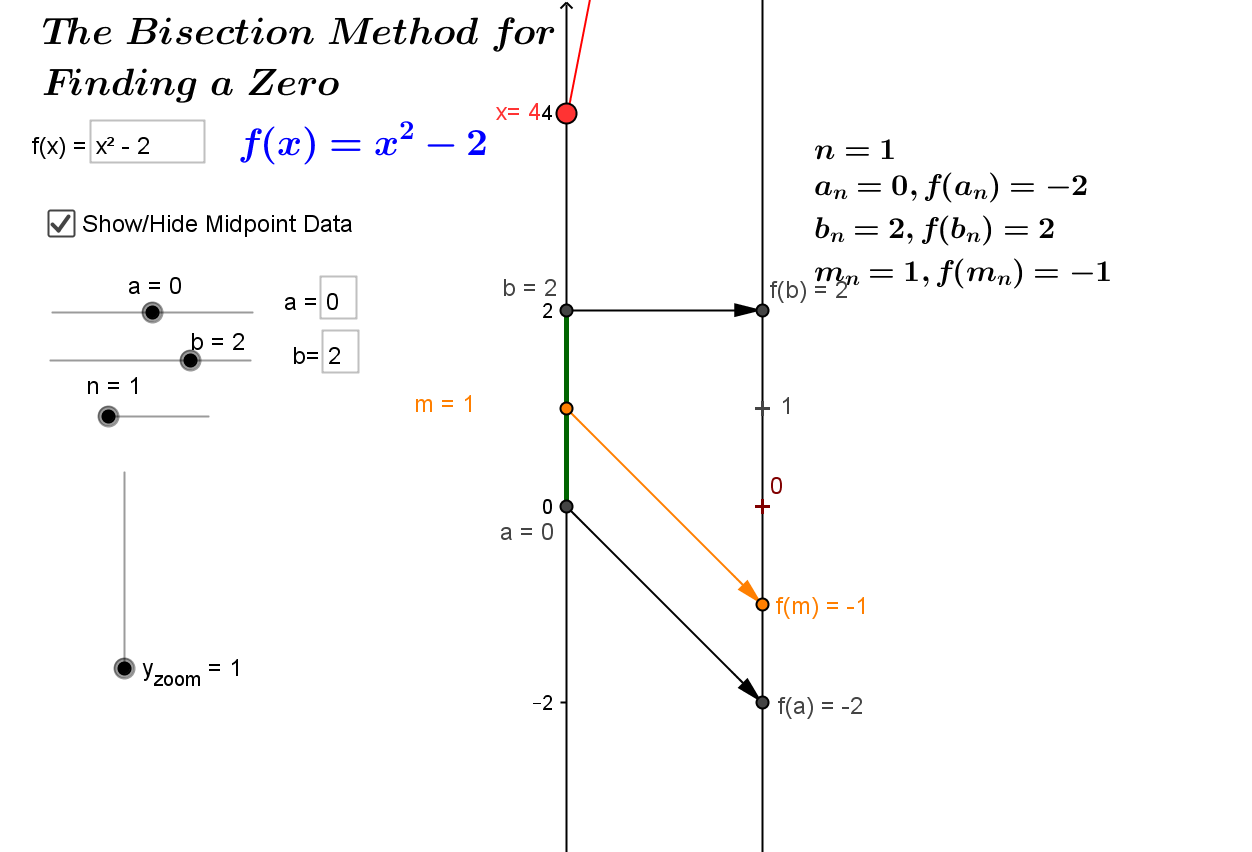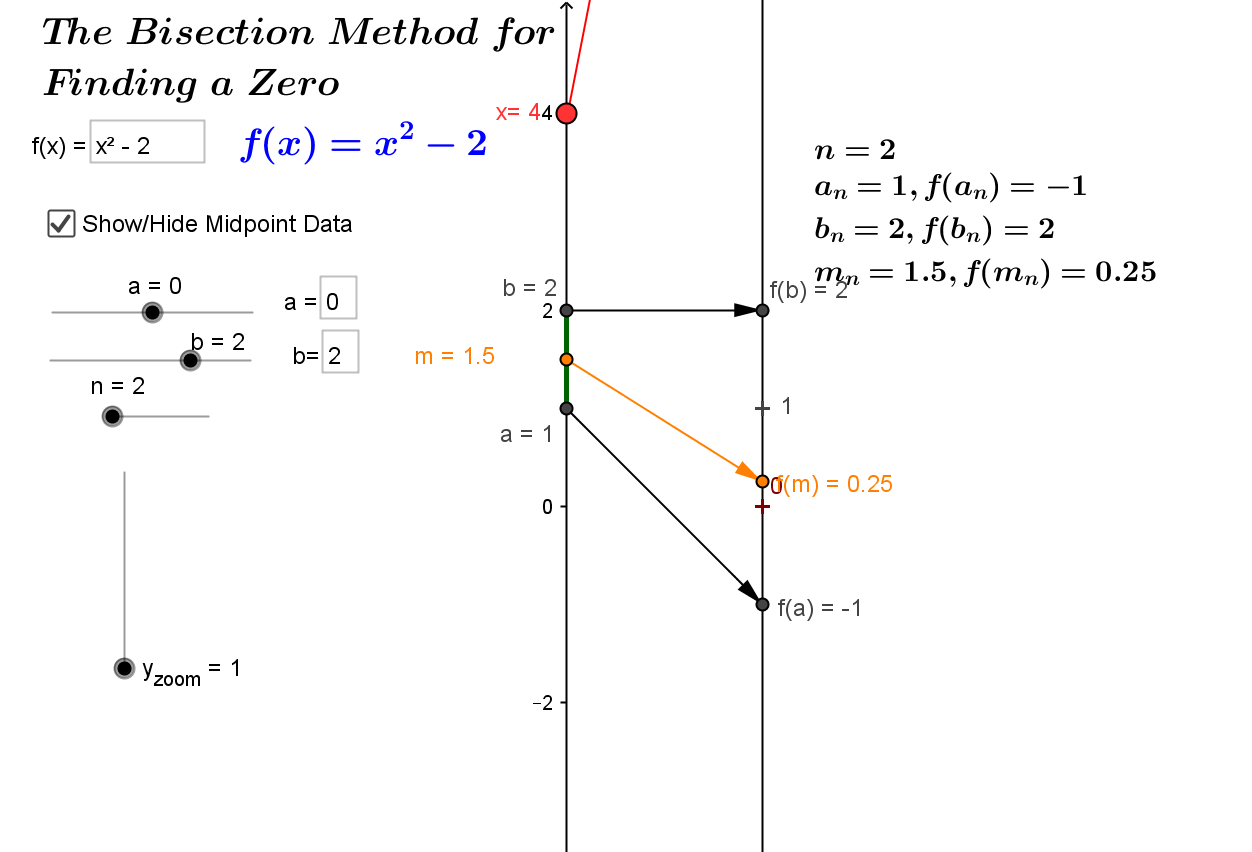Bisection and Proof Outline of IVT vizualized with GEOGEBRA.
This is a Java Applet created using GeoGebra from www.geogebra.org - it looks like you don't have Java installed, please go to www.java.com

## Axioms for the Real numbers- from A first Analysis course by John O'Connor

What distinguishes R from Q (and from other subfields of R) is the Completeness Axiom.

Definitions:
An upper bound of a non-empty subset A of R is an element b in R with b ge a for all a in A.
An element M in R is a least upper bound or supremum of A if
M is an upper bound of A and if b is an upper bound of A then b ge M.

A  lower bound of a non-empty subset A of R is an element b in R with b le a for all a in A.
An element m in R is a greatest lower bound or infimum of A if
m is an lower bound of A and if b is a lower bound of A then b le m.

The Completeness Axiom

If a non-empty set A has an upper bound, it has a least upper bound.

Look at Exercises 3 from A first Analysis course by John O'Connor

Motivation for believing Completeness Axiom:
Using decimals to find decimal places of sqrt{ 3}:
Start with 1^1 = 1 and 2^2=4, so the units decimal for sqrt{ 3} is 1.
Now to find the tenths decimal digit consider
1^2, 1.1^2 , 1.2^2, 1.3^2, ...,1.7^2 = 2.89 , 1.8^2 = 3.24
, 1.9^2, 2^2.
So the tenth digit is 7.
To find the hundredth digit consider
1.70^2, 1.71^2 , 1.72^2, 1.73^2 = 2.9929, 1.74^2= 3.0276, ... , 1.78^2, 1.79^2, 1.8^2.

So the hundredth digit is 3.
Continue in this fashion, for each decimal place, examine the eleven numbers that might have the correct digit, and choose the digit where the squared value changes from being smaller than 3 to being larger than 3.

Now prove the infinite decimal that results must have its square equal to 3. ...

Fact: If L and L' are least upper bounds for a set S, then L = L'.
Proof: Since L' is an upper bound for S and L is a least upper bound for S, L <= L'. Likewise, L' <= L. So L = L'. (trichotomy property) EOP.

Credibility without proof for the Completeness:
We can use decimals, representing real numbers, to help give credibility to the belief that the Completeness Property is true for the real numbers.

Discussion: Since S is nonempty, there is an x_0 in S. Suppose B is an upper bound for S, so B>=x_0. If B = x_0 then it is not hard to show that B is the least upper bound  of S.

So,we suppose B>x_0. Now there is an integer m_0 with m_0 > B and an integer n_0 with n_0 < x_0.  [Why?]

In the interval [n_0,m_0] there is an integer m_1 where m_1 is an upper bound for S but m_1 -1 is not an upper bound for S. If m_1 in S  then m_1 is the least upper bound of S.  Otherwise let n_1 = m_1-1 and now consider the interval [n_1,m_1].

In the interval [n_1,m_1] there is a decimal n_1 +k_1*0.1 where 0< k_1 le 10 is an integer and n_1+k_1*0.1 is an upper bound for S but n_1+ (k_1-1)*0.1 is not an upper bound for S.

Let m_2 = n_1 +k_1*0.1
. If m_2 in S  then m_2 is the least upper bound of S.
Otherwise,  let n_2 = n_1 +(k_1-1)*0.1 and now consider the interval  [n_2,m_2].

In the interval [n_2,m_2] there is a decimal n_2 +k_2*0.01 where 0< k_2 le 10 is an integer and n_2+k_2*0.01 is an upper bound for S but n_2+ (k_2-1)*0.01 is not an upper bound for S.
Let m_3 = n_2 +k_2*0.01. If m_3 in S  then m_3 is the least upper bound of S.
Otherwise,  let  n_3 = n_2 +(k_2-1)*0.01 and now consider the interval [n_3,m_3].

We can continue in this fashion. After each step that continues we obtain a "decreasing" sequence of terminating decimals m_j which are upper bounds for S and an "increasing" sequence of terminating decimals n_j each of which is just one digit smaller in the last decimal place than m_j and each of which is not an upper bound for S .
These decimals allow us to determine a number to any decimal precision which approximates the least upper bound L.  L will be the limit of the two sequences n_j and m_j.

The indirect proof that L is the least upper bound of S would involve an argument assuming either that L was not an upper bound for S  or that there was an upper bound of S that was smaller than L  and consideration of how the two sequences were determined. This argument is omitted
here as this argument is given only to suggest the truth of the completeness property for real numbers.

## Rethink proof of The intermediate value theorem (IVT) using the LUB property.

Assume  that f is continuous on the interval [a,b] and f(a) < 0 < f(b). The theorem concludes that there is a number c in (a,b) with f(c) = 0.
For the purpose of this "proof" we interpret "continuous at  t " as meaning that when x ~~ t
then f(x) ~~ f(t).

 The Plan: Use the completeness property for a subset of [a,b] to find a candidate for c and then show that f(c) =0 by showing that f(c) cannot be positive or negative, leaving f(c) = 0 as the only possibility. The Set: Let X = \{ x in [a,b]: for all y in [a,x], f(y) le 0 \}. X is not empty since f(a) <0, and thus there is some small interval [a, a+epsilon] where by continuity f is negative for all a le x le a +epsilon. X is bounded above by b, so by  the completeness of the real numbers, it has a least upper bound, which we denote c. From the remark that showed that X is not empty, it also follows that a0 there is some small interval [b - epsilon, b] where by continuity f is positive for all b -epsilon le x le b. Thus c 0 are both impossible, leading to the conclusion that f(c) = 0. This is a Java Applet created using GeoGebra from www.geogebra.org - it looks like you don't have Java installed, please go to www.java.com

(i) Suppose f(c) < 0. Then by continuity, there is an interval, I, containing c where f(y)< 0 for all y in I.
In I there is some s with s<c, so s is not an upper bound for X. Thus there is some x* in X with s<x*<c.
Notice that there is some r with r in I and c< r.
Considering [a,x*] and [x*,r] we have that for all y in [a,r], f(y) le 0. Thus r in X. This contradicts that c is an upper bound for X, so  f(c) < 0 is false.

(ii) Suppose f(c)>0. Then by continuity, there is an interval, J, containing c where f(y)> 0 for all y in J.
But then there is some s with s<c,  with  f(s) > 0. So if  x in X then x < s and thus  s is an upper bound for X smaller than c. This contradicts that c is the least upper bound of X, so f(c) >0 is false

Since (i) and (ii) have eliminated f(c) < 0 and f(c) > 0 as possibilities, it must be that f(c) =0.    EOP

2-8
• Motivational Question I: What is a number?
• Motivational Question II: What does continuity mean?
• Motivational Question III: What does limit mean? for numbers? for function values? for functions?  for sets?

GeoGebra Applet for visualizing  sequences with Mapping Diagrams and Graphs for a sequence defined by a_n = f(n). Examples.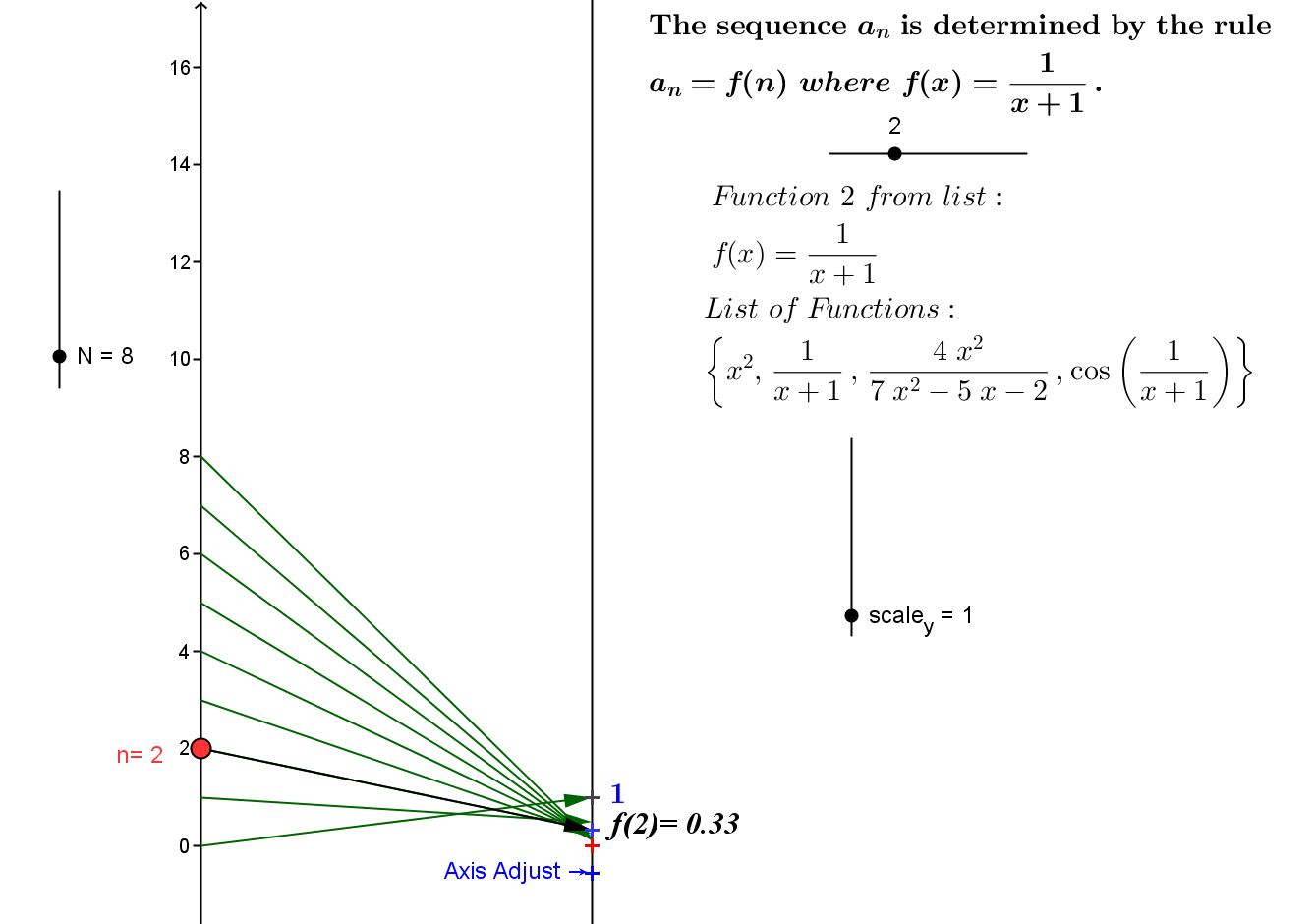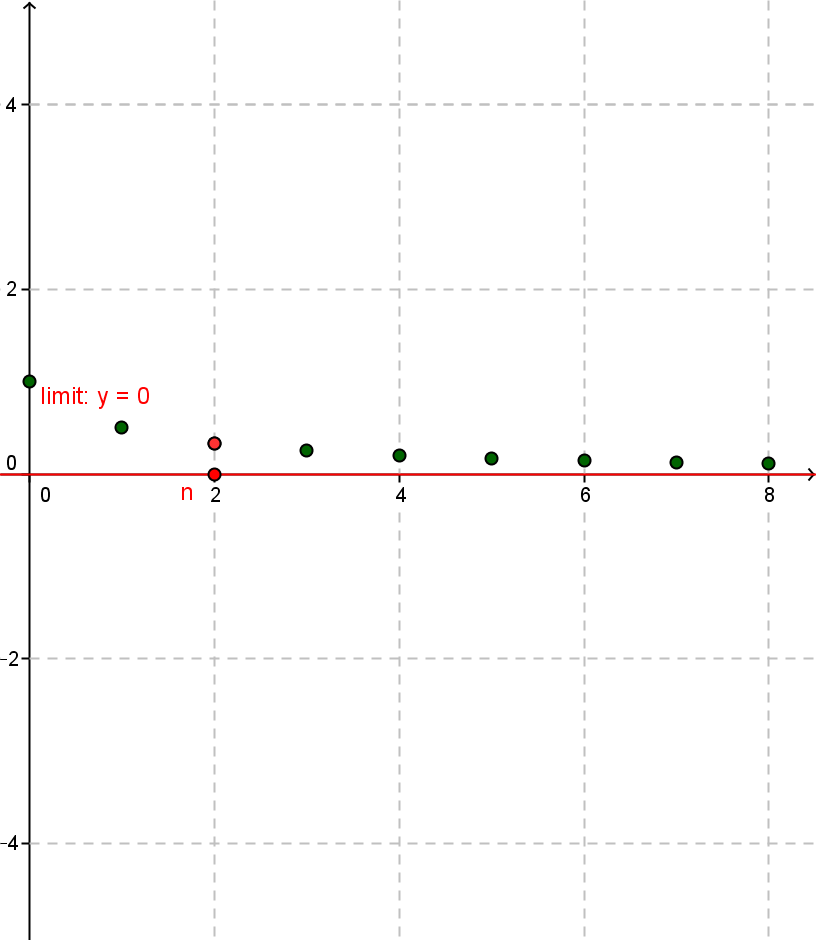This is a Java Applet created using GeoGebra from www.geogebra.org - it looks like you don't have Java installed, please go to www.java.com

GeoGebra Applet for visualizing  sequences with Mapping Diagrams and Graphs for a sequence defined by a_n = f(n).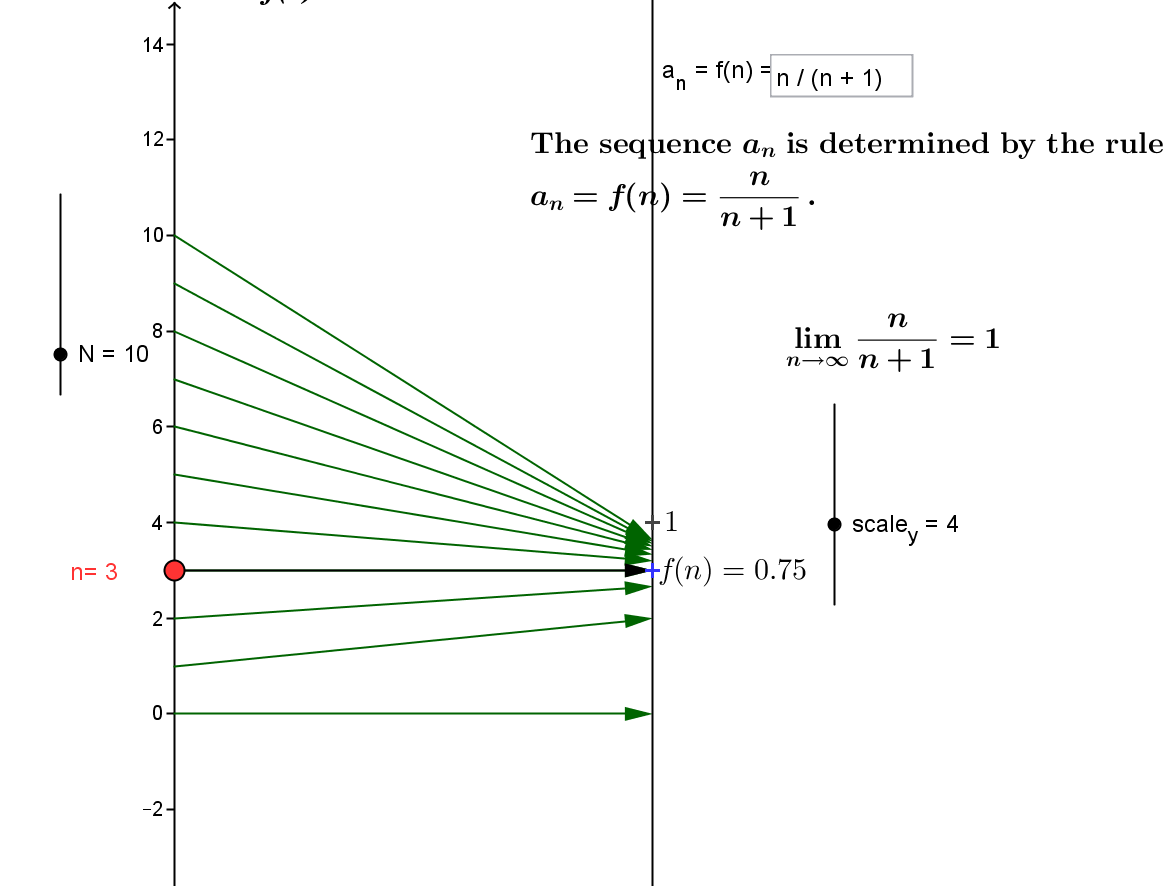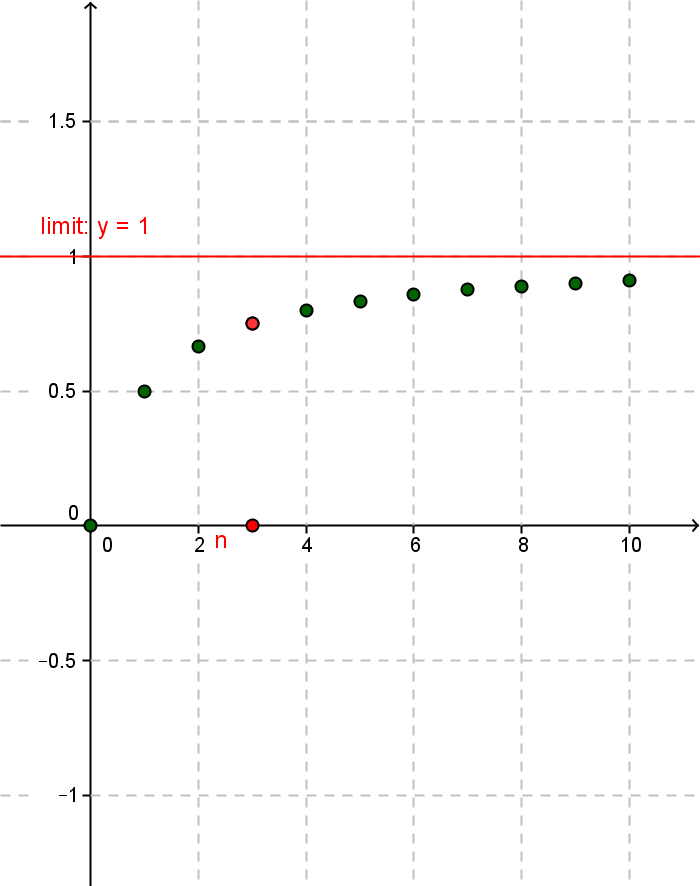This is a Java Applet created using GeoGebra from www.geogebra.org - it looks like you don't have Java installed, please go to www.java.com

• Provisional Definition: limit  of  a_n is a means "after a while all a_n are close to a."
2-9
• Def'n: We say lim_{n->oo} a_n = a if
given any epsilon >0 there is a natural number M so that
if n>M then | a_n - a | < epsilon.
Comment: What kind of number is epsilon?
• Visualizing the definition of  lim_{n->oo}a_n = a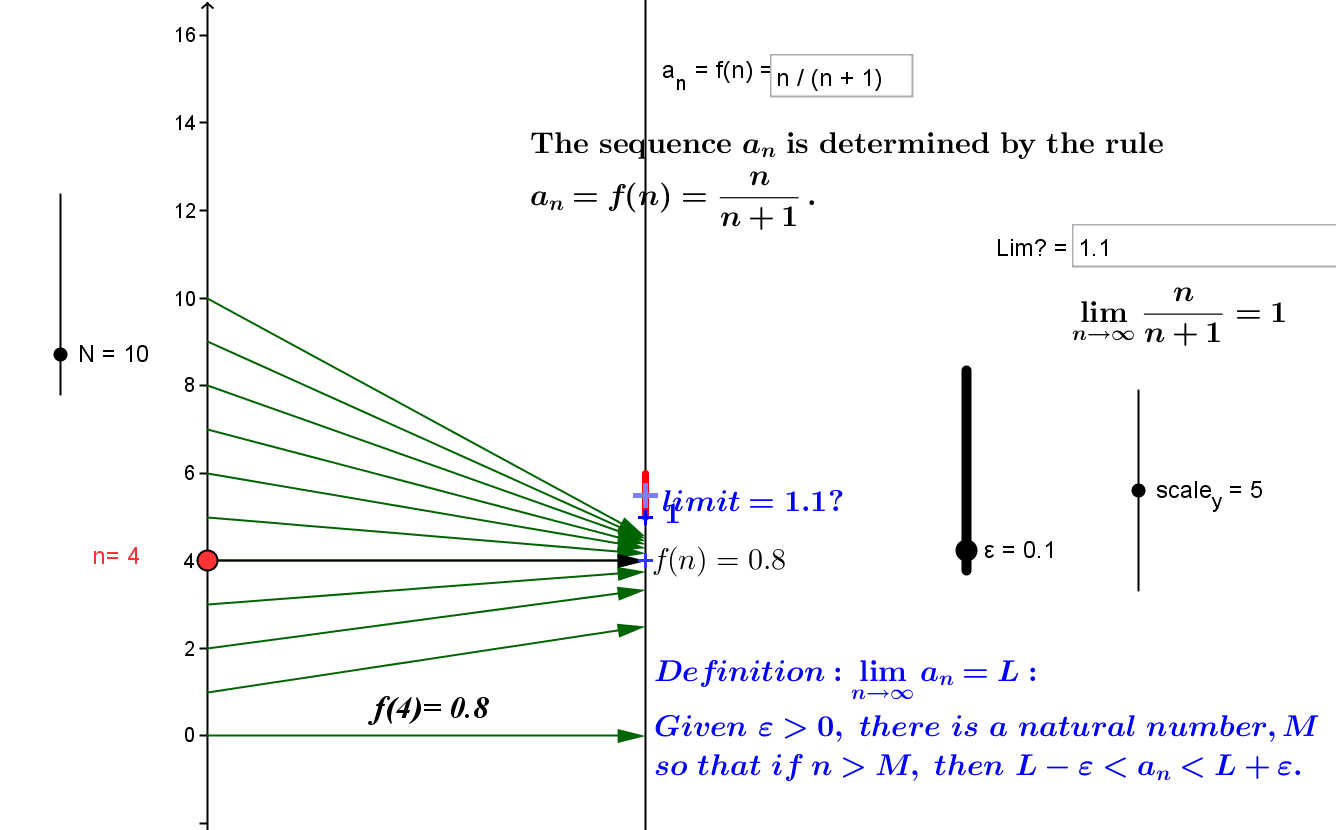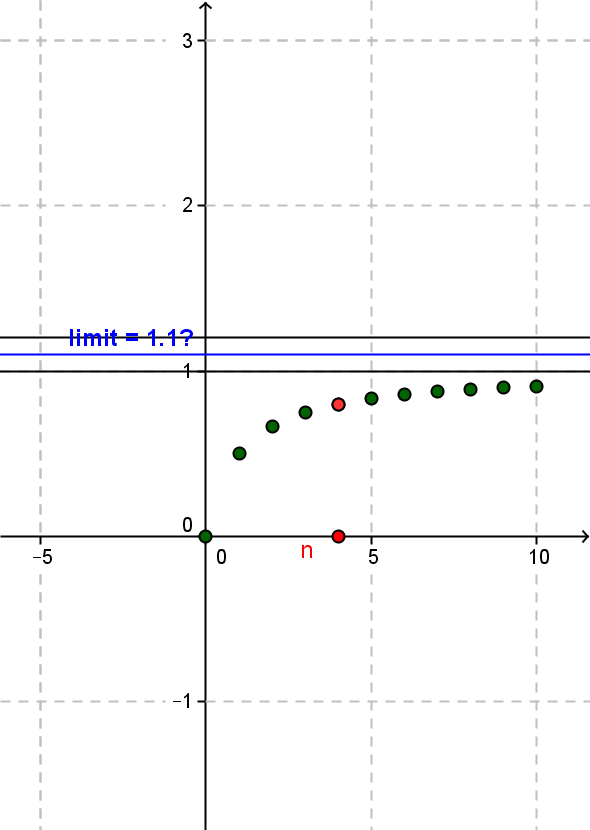Example: lim_{n->oo} 1/n = 0.
Proof: Suppose epsilon >0. Then by the Archimedean Property there is a natural number M  where 1/M < epsilon. To show this M works, suppose n > M. Then we have 1/n < 1/M [Prove this---?] . Thus |1/n - 0| = 1/n < 1/M < epsilon. EOP.

This is a Java Applet created using GeoGebra from www.geogebra.org - it looks like you don't have Java installed, please go to www.java.com

2-11
Example:
Show that lim_{n->oo}n/{n+1} is not 1.1.
Solution: Supose lim_{n->oo}n/{n+1}=1.1 . Then let epsilon = 0.05 and consider any natural number n. Then n/{n+1} lt 1 lt 1.1 and |n/{n+1}-1.1| gt |1-1.1| =0.1 > 0.05. So for any natural number M, if  n >M then |n/{n+1}-1.1|  > 0.05 which contradicts the definition of limit which says that for any epsilon >0 there is a natural number M so that if n>M then | a_n - a | = |n/{n+1}-1.1| < epsilon.

Example: Show that lim_{n->oo}(-1)^n does not exist.
Solution: Supose there is a number L where lim_{n->oo}(-1)^n = L . Then let epsilon = 0.5.  So if  lim_{n->oo}(-1)^n = L then there is a natural number M, where if  n >M then |(-1)^n - L| < 0.5 or L-0.5 < (-1) ^n < L +0.5. Consider- if n is even then (-1)^n = 1 so L-0.5 < 1 < L +0.5 and 0.5 <L < 1.5. While if n is odd the (-1)^n = -1 and L-0.5 < -1 < L +0.5 so -1.5<L < -.5. But then  L is both positive and negative - which is a contradiction.

Proposition: [ "Limits are unique."] If  lim_{n->oo} a_n = A and lim_{n->oo} a_n = B then A = B.
Proof: Suppose
lim_{n->oo} a_n = A and lim_{n->oo} a_n = B.
Let  epsilon = {|A-B|}/3.
By the definition there is a natural number M_A where  if n>M_A then | a_n - A | < epsilon.
Likewise there is a natural number M_B where  if n>M_B then | a_n - B | < epsilon.
Now let n = M_B + M_A +1 so that n > M_A  and n > M_B.
Then | a_n - A | < epsilon=  {|A-B|}/3 or  A - {|A-B|}/3 < a_n <A +  {|A-B|}/3.
Similiarly
B - {|A-B|}/3 < a_n <B +  {|A-B|}/3.

If  B<A then  these inequalities say {2 A +B}/3 < a_n< {4A - B}/3 while {4B - A}/3 < a_n < {2B + A}/3 .
But this means {2 A +B}/3 < a_n < {2B + A}/3  which implies 2 A +B <  2B + A  or A <B.  A contradiction!
Similarly B>A also leads to a contradiction. So it must be that A = B.

For a few more examples see: Convergence in the Reals

2-12
Motivation:
Preview of definition for limit of a function and continuity based on sequence limits.
Suppose a in domain of  f.
We say lim_{x->a} f(x) = L if for any sequence a_n in the domain of f -{a}
with lim_{n->oo}a_n = a,  lim_{n->oo} f(a_n) = L.

We say f  is continuous on its domain D if for any a in D and  for any sequence a_n in the domain of f where lim_{n->oo}a_n = a,  lim_{n->oo} f(a_n) = f(a) or
lim_{x->a} f(x) = f(a)

This is a Java Applet created using GeoGebra from www.geogebra.org - it looks like you don't have Java installed, please go to www.java.com

Theorems connected to algebra, inequalities and intervals.
Proof: Suppose {a_n}→ a and {b_n}→ b and  we are given epsilon > 0.
[Plan:We need to find a natural number M so that if n>M, we can show that |a_n+b_n-(a+b)| < epsilon. We will use the convergence of a_n and b_n to find M.]
For the sequence \{a_n\} there is a natural number M_a where if n>M_a we have that |a_n-a|< epsilon/3 or a-epsilon/3 < a_n < a + epsilon/3 .

Similarly for the sequence \{b_n\} there is a natural number M_b where if n>M_b we have that |b_n-b|< epsilon/3 or b-epsilon/3 < b_n < b + epsilon/3.
Now let M = M_a +M_b +1.

Then for n > M, n > M_a and n> M_b so
a-epsilon/3 < a_n < a + epsilon/3 and b-epsilon/3 < b_n < b + epsilon/3.

a+b- 2epsilon/3 < a_n +b_n <a + b + 2epsilon/3 or | a_n +b_n - (a+b) | < 2 epsilon/3 < epsilon. EOP.

2-15
Class started with discussion of Problem 3 of assignment due Feb. 8.
Work on understanding the problem led to specifying the function as f(x) = x^2 and intervals [-1/2, 1/3] and [1,2] and the set {3,5,sqrt 2}. Discussion considered the graph and mapping diagram and how the extreme value theorem and intermediate vlue theorems were used to find intervals and number to describe f(D).

Reference was made to the following proof for (i).
Alternative Proof: Choose M = max \{M_a,M_b\}.
Then for n > M, n > M_a and n> M_b so
|a_n + b_n - (a+b)| = |(a_n - a)  + (b_n - b)| le |a_n - a|  + |b_n - b| < epsilon/3 + epsilon/3= 2 epsilon/3 < epsilon. EOP.

(ii) (an- bn)→ a - b
Proof: Leave till after (iii). Then apply i) and iii) to
(an+ (-1) bn) → a +(-1) b = a - b.

2-16
(iii)
(anbn)→ a b

Proof: Suppose {a_n}→ a and {b_n}→ b and  we are given epsilon > 0.
[Plan:We need to find a natural number M so that if n>M, we can show that |a_n b_n-(ab)| < epsilon.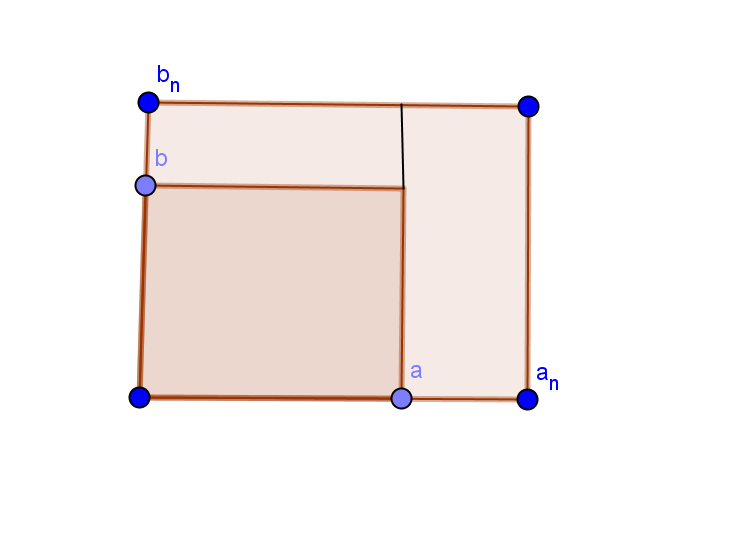We will use the convergence of a_n and b_n  and the fact that convergent sequences are bounded to find M. Also we will  consider these products as areas of two rectangular regions: one with sides a and b and and the other with sides a_nand b_n.]
This visualizes the algebra that |a_n b_n - ab| = |(a_n - a)b_n + a(b_n - b)|.
Let B>0 with B> |b_n| for all n. [Explain why this is possible.]
Let A >0 so that A>|a|  so 0 le |a|/A < 1.

For the sequence \{a_n\} there is a natural number M_a where if n>M_a we have that |a_n-a|< epsilon/{3B}.
F
or the sequence \{b_n\} there is a natural number M_b where if n>M_b we have that |b_n-b|< epsilon/{3A}.
Choose M = max \{M_a,M_b\}.
Then for n > M, n > M_a and n> M_b so
|a_n b_n - ab| = |(a_n - a)b_n + a(b_n - b)| le |a_n - a||b_n|  + |a| |b_n - b| < epsilon/{3B}B + |a| epsilon/{3A}< 2 epsilon/3 < epsilon. EOP.

(iv) (an/bn)a/b (provided bn ≠ 0 and b ≠ 0).
Lemma:
1/{b_n} \to 1/b  (provided bn ≠ 0 and b ≠ 0).
Proof of lemma:
Suppose {b_n}→ b ne 0 and  we are given epsilon > 0.
[Plan:We need to find a natural number M so that if n>M, we can show that |1/ {b_n}-1/b| < epsilon. We will use the convergence of  b_n, b_n ne 0, b ne 0  and the fact that convergent sequences are bounded to find M.]
Consider that
by (iii) the sequence {b_n b} converges to b^2 ne 0.
From this it can be shown (an exercise for the reader) that there is a number B
so that |b b_n|> B>0 and B/{b b_n} < 1 for all n.
For the sequence \{b_n\} there is a natural number M_b where if n>M_b we have that |b_n-b|< B*epsilon/2. Then for n > M_b we have

|1/{b_n} - 1/b| = |{b- b_n}/{b b_n}|  =  {|b- b_n|}/{|b b_n|} <  epsilon B/{2 |b b_n|}<  epsilon/2 < epsilon. EOP.

Application of arithmetic for sequences to limits and continuity of functions:
Example: Let f(x) = x^2. Prove that lim_{x \to 3} f(x)= 9 [ which = f(3)].
Demonstration: Referring to the limit definition for a function we suppose that we have a sequence  {a_n} with a_n ne 3 and lim_{n \to oo} a_n = 3. Then we consider the sequence {f(a_n) = a_n^2} and apply (iii)  so that
lim_{n \to oo} a_n^2 = lim_{n \to oo} a_n cdot a_n = lim_{n \to oo} a_n cdot  lim_{n \to oo} a_n = 3 cdot 3 = 9.
Notes: 1. This example shows the function f(x) = x^2 is continuous at x = 3.
It is not difficult to show then that this function
f(x) = x^2 is continuous for any a, and so is continuous for the domain (-oo, oo).
2.
Let g(x) = x^2 when x ne 3 and g(3) = 7. Prove that lim_{x \to 3} g(x) = 9 [ which  ne f(3)].
Demonstration: Referring to the limit definition for a function we suppose that we have a sequence  {a_n} with a_n ne 3 and lim_{n \to oo} a_n = 3. Then we consider the sequence {g(a_n) = a_n^2} and apply (iii)  so that
lim_{n \to oo} a_n^2 = 9. Here the limit exists as x approaches 3, but the limit is not equal to g(3) =7, so g is not continuous at x=3.
3. Similar arguments can show that if f(x) = b_0 +b_1x+b_2x^2 + ...+b_nx^n and  g(x) = c_0 +c_1x+c_2x^2 + ...+c_mx^m
then as long as g(a) ne 0, the function h(x) = {f(x)}/{g(x)} is continuous at x=a.

2-18
For Friday class discussion:
Theorem: Suppose f and g are functions with domains (-oo,oo), h(x) = g(f(x)) for all x,  f  is continuous at x=a and g is continuous at b = f(a). Prove h is continuous at x=a.
Here is a GeoGebra mapping diagram to help you visualize the theorem.

This is a Java Applet created using GeoGebra from www.geogebra.org - it looks like you don't have Java installed, please go to www.java.com

The Squeeze Lemma (for sequences): If a_n <= c_n <= b_n  for all n and lim_{n->oo}a_n = c and lim_{n->oo}b_n = c, then lim_{n->oo}c_n = c.
Proof: Suppose the hypotheses and that we are given epsilon > 0.
[Plan: We need to find an M so that if n > M, then |c_n - c| < epsilon.  Connect |a_n-c| and  |b_n -c| with
a_n <= c_n <= b_n  and the limit assumptions |c_n-c|]
Since  a_n <= c_n <= b_n ,  a_n -c <= c_n -c <= b_n -c  and this  0 <= |c_n -c| <= max \{|b_n -c|, |a_n -c| \}
For the sequence \{a_n\} there is a natural number M_a where if n>M_a we have that |a_n-c|< epsilon.
For the sequence \{b_n\} there is a natural number M_b where if n>M_b we have that |b_n-c|< epsilon.
Let M= max\{ M_a,M_b\} and then ... [Finish the argument.]
Class Exercise: State and prove the squeeze lemma (for functions).

2-19
Theorem: Suppose f and g are functions with domains (-oo,oo), h(x) = g(f(x)) for all x,  f  is continuous at x=a and g is continuous at b = f(a). Prove h is continuous at x=a.
Proof: Suppose {a_n} with lim_{n to oo} a_n = a. By continuity of f at x=a we have that  lim_{n to oo} f(a_n) = f(a)= b.  By continuity of g at x=b we have that  lim_{n to oo} g(f(a_n)) = g(f(a))= g(b) = h(a).  Thus  we have that  lim_{n to oo} h(a_n) = h(a), showing that h is continuous at x=a.

Monotonic sequences
Theorem BMC:
A bounded monotonic (increasing) sequence is convergent.
Proof: Suppose {a_n}  is a sequence with  a_n le a_{n+1}  and a_n < B for all n.
By the completeness of the real numbers let L be the least upper bound of  {a_n}. Claim : lim_{n to oo} a_n = L. Here's why: Suppose epsilon > 0. Then L- epsilon < L and hence L - epsilon is not an upper bound for  {a_n}. So there is a natural number M where L - epsilon < a_M < L and so for n > M we have L - epsilon < a_M < a_n < L or | a_n - L | < epsilon.
Comment: This result gives a sufficient condition for a sequence to have a limit without knowing in advance what that limit is. The proof finds the limit by using the completeness of the real numbers.
The Bolzano Weierstass Theorem: Every bounded sequence has a convergent subsequence.
The proof of BWT can be developed using Spivak's lemma:
Lemma: Every (bounded) sequence has a monotone subsequence.
Proof: (Spivak) ... Two cases:
 Case i) There are an infinite number of n with the property that if m>n then a_m < a_n. Put these  n's in order, so n_1< n_2<... and a_{n_1} > a_{n_2}> ... and we havefound a subsequence that is monotonic decreasing.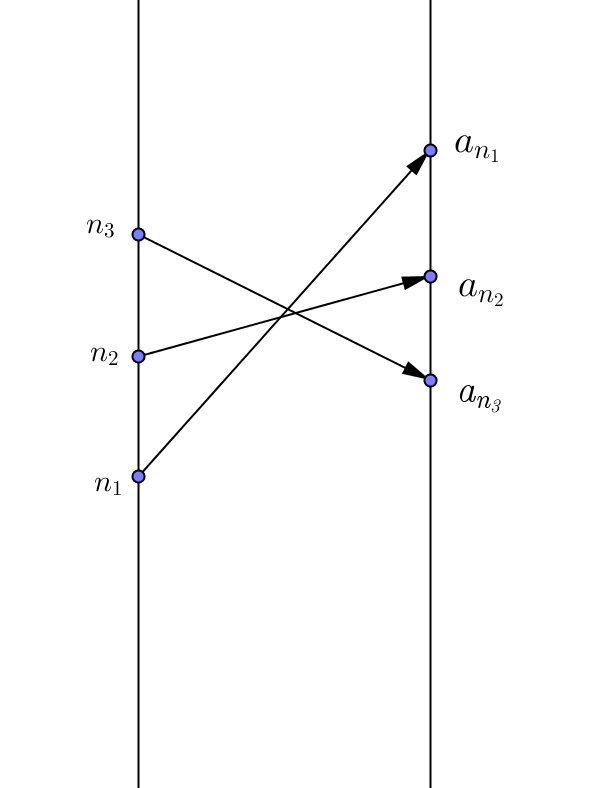Case ii) There is a largest number N with the property that if m>N then a_m < a_N.  Then let n*_0 = N+1> N. Then there is a number n*_1 > n*_0 where a_{n*_1} ge a_{n*_0}. We can continue to create sequence of  n*'s where a_{n*_{k+1}} ge a_{n*_k} and we have found a subsequence that is monotonic increasing.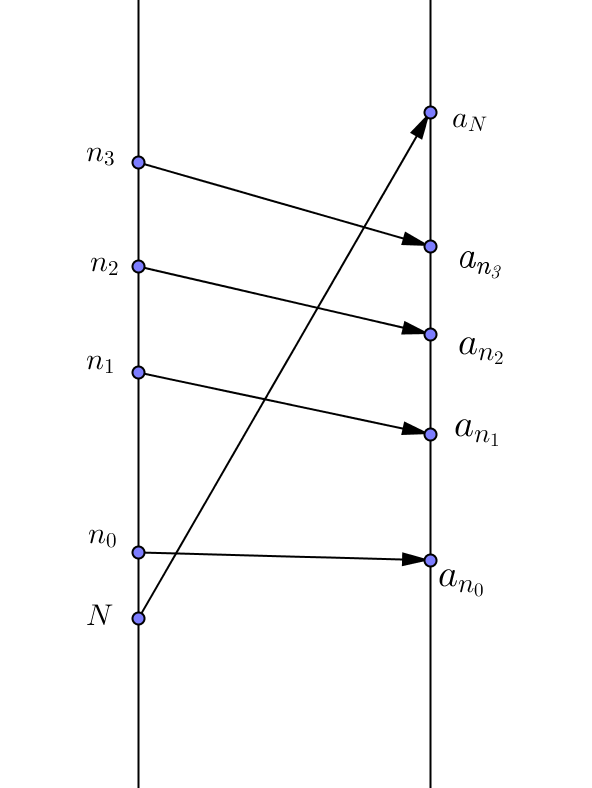EOP.

Proof of BWT: Suppose {a_n} is a bounded sequence. Then it has a monotonic subsequence which is still bounded. This subsequence is convergent by the BMC theorem.

2-22
Cauchy criterion for convergence:

Definition

A sequence ${an}$ is called a Cauchy sequence if the terms of the sequence eventually all become arbitrarily close to one another.
That is, given
$ε$
> 0 there exists N such that if m, n > N then |am- an| < $ε$.
Proposition: If $an$ converges, then $an$ is a Cauchy sequence.
Proof: Suppose $limn→∞an=L$, then given $ε>0$
, there exists N such that if n > N then |an - L| < $ε2$. Thus if $m,n>L$ then .

Theorem (CC): If ${an$} is a Cauchy sequence, then ${an$} converges.
Proof  Plan: 1 . Show ${an$} is bounded.
2. Use the B. W. Theorem to give a subsequence of ${an$} that converges to $L$.
3. Show $limn→∞an=L$.

For more details of the proof see Cauchy criterion for convergence

2-23
Some "monster" examples of functions and continuity
Note: Between any two rational numbers there is an irrational number.

Between any two irrational numbers there is a rational number.
Discussed extensively in class using the decimal estimation of a irrational numbers and sqrt 2 for finding an irrational number between two rational numbers.

"Density"
Proposition: If a and b are rational numbers with a < b, then there is an irrational number c with a < c < b.
Proof: Using the linear function f(x) = a + (b-a)(x-1) we see that f(1) = a, f(2) = b, and if  1<x<2 then a<f(x) <b.
Since 1< sqrt{ 2}< 2, we have c = f(sqrt{ 2}) in (a,b).
But if c is a rational number  then   c = a + (b-a)(sqrt{ 2} -1)  is a rational number.
Solving for sqrt{ 2} we have sqrt{ 2} = {c-a}/{b-a} + 1, a rational number. This contradicts the fact that sqrt{ 2} is not a rational number, so c is not a rational number, i.e., c is an irrational number.                EOP.

Proposition: If a and b are irrational numbers with a < b, then there is an rational number c with a < c < b.
Proof: Suppose a and b are irrational numbers with a<b. Treating these as infinite decimals a= n_a + .a_1a_2a_3...  and b= n_b + .b_1b_2b_3.... there is some decimal position where these two numbers differ. If n_a<n_b then since b is not a rational number a<n_b<b and we are done. Id n_a= n_b let k be the smallest natural number where a_k<b_k.  Then let c = n_a+ .a_1a_2...b_k00000.... Then a< c<b.  EOP.

Remark: In fact-- If a and b are real numbers with a < b, then there is an rational number c_0 with a < c_0 < b and there is an irrational number c_1 with a < c_1 < b.

Examples:

1. f(x) = 1 for x le 5, f(x) = 0 for x> 5.
f is continuous for all x ne 5. f is not continuous at  x = 5. Consider a_n = 5+1/n; n = 1,2,.... and note that lim_{n to oo} f(a_n) = 0 ne 1 = f(5).
2. f(x) = 1 for x a rational number, f(x) = 0 for x an irrational number.
f is not continuous at any  x = a.
Consider when a is irrational a_n the nth truncated estimate of a as a decimal; n = 1,2,.... and note that lim_{n to oo} f(a_n) = 1 ne 0 = f(a).
Consider when a is rational a_n = a+ {sqrt 2}/n; n = 1,2,.... and note that lim_{n to oo} f(a_n) = 0 ne 1 = f(a).
3. f(x) = 1/q for x = p/q where gcd(p,q) = 1, f(x) = 0 for x an irrational number.
4.  Let f(x) = x if  x is rational and f(x) = 0 if  x is irrational.
Then f is continuous at x=0 but not continuous at any other real number.

The intermediate value theorem (again!).
I V Theorem [0 version]: If $f$ is continuous on $[a,b]$ and then there is a number $c∈(a,b)$ with $f(c)=0$.
Proof: Plan. Create  sequences $an$ and $bn$ where   and for any $n$, and $|an-bn|=b-a2n$.
Then both sequences are monotonic and bounded and converge to the same number $c∈(a,b)$. Using continuity show that $f(c)=0$.

Here's how  to construct the $an$ and $bn$. [The basic idea is bisection.]

Let $a0=a$ and $b0=b$.  Now let $m0=a0+b02.$
If $f(a0)⋅f(m0)=0$, we have found the desired $c=m0$ for the theorem... stop here. :)

If $f(a0)⋅f(m0)>0$, let $a1=m0$ and $b1=b0$.
If , let $a1=a0$ and $b1=m0$.
Then and and  $|a1-b1|=b-a2$.

Continue in the same way to construct $an+1$ and $bn+1$ from $an$ and $bn$:
Now let $mn=an+bn2.$
If $f(an)⋅f(mn)=0$, we have found the desired $c=mn$ for the theorem... stop here. :)

If $f(an)⋅f(mn)>0$, let $an+1=mn$ and $bn+1=bn$.
If , let $an+1=an$ and $bn+1=mn$.
Then and .

Continuation of proof :
Now the sequences ${an}$ and ${bn}$ are monotonic and bounded, so they each have a limit, call them $ca$ and $cb$
Noting that  $|an-bn|=b-a2n$ it is an exercise for the reader to show that $ca=cb$.

We let $c=ca=cb$ and claim that $f(c)=0.$
Since $f(an)⋅f(an+1)>0$ for all $n$, we can say the limit of  $f(an)$ is $f(ca)$ by continuity , and  thus  $f(a)⋅f(ca)≥0$.
Likewise, since $f(bn)⋅f(bn+1)>0$ for all $n$, we can say the limit of  $f(bn)$  is $f(cb)$ by continuity , and thus $f(b)⋅f(cb)≥0$.

But since $ca=cb=c$,
we have $f(a)⋅f(c)≥0$ and $f(b)⋅f(c)≥0$,
and thus by multiplication, $f(a)⋅f(c)⋅f(b)⋅f(c)≥0$ ($□$)

But from the hypothesis, ,  and $f(c)⋅f(c)≥0$.
If $f(c)⋅f(c)>0$ then  , a contradiction of ($□$)
Thus $f(c)⋅f(c)=0$ and $f(c)=0$.   EOP.

Cor. I V Theorem [general version]: If $f$ is continuous on $[a,b]$ and $v$ is between $f(a)$ and $f(b)$  then there is a number $c∈(a,b)$ with $f(c)=v$
Proof: Consider  $h(x)=f(x)-v$ for all $x∈[a,b]$. Then $h$ is continuous on $[a,b]$ and , so $h$ satisfies the hypotheses of IV T[0 -version], so there is a number $c∈(a,b)$ where $h(c)=f(c)-v=0.$ Thus $f(c)=v$. EOP.

2-25
Begin with proof of the extreme value theorem.
The boundedness theorems.

2-26
More on horrible functions from previous class:

1. f(x) = 1/q for x = p/q where gcd(p,q) = 1, f(x) = 0 for x an irrational number.
Result: f is not continuous at any rational number, x = p/q. Let a_n =x + sqrt 2 then lim_{n to oo} a_n =x and f(a_n) =0 so
lim_{n to oo} f(a_n) = 0 ne 1/q for x = p/q, gcd(p.q)=1.
f is continuous for all x, where x is irrational.

2.  Let f(x) = x if  x is rational and f(x) = 0 if  x is irrational.
Then f is continuous at x=0 but not continuous at any other real number.

Review of Rational Numbers  Q and Irrational Numbers, R-Q:

Def'n. A number x is a rational number if there exist integers n and m with m \ne 0 where x = n/m.
A number x is a d-rational number if there exists an integer q and a sequence {d_i}_{i=1,2,...} where d_i in N,  0<=d_i<=9
with x=q +.d_1d_2 ... = q + \sum_{i=1}^{oo) d_i*10^{-i},
and
there exist M and r in N where if i>=M then d_{i+r}=d_i.

Examples:
3/5 and {-11}/7 are rational numbers. sqrt{3} is not a rational number.
57.4368686868... is a d-rational number, q =57,  d_1=4, d_2 = 3, d_3=6, d_4=8, d_{3+2j}=6, d_{4+2j}=8 for  j in N.  M=3, r=2.

Theorem: x is a rational number if and only if x is a d-rational number.
Proof outline:
=>: Suppose x is a rational number,  x= n/m  with m,n in Z and m > 0. Do the "long division".  By the division algorithm for natural numbers, the remainders R in N are always 0<= R < m, so eventually the long division will give repeating blocks of digits and this shows x is an d- rational number.
\Leftarrow:  Suppose x is a d- rational number, x=q +.d_1d_2 ... = q + \sum_{i=1}^{oo) d_i*10^{-i}. Then consider 10^r x - x = (10^r -1)*x  a terminating decimal, which shows that x  can be recognized as a rational number by some simple arithmetic.

More examples of some horrible functions for limits and continuity, including the mathematician's sine.
Examples: i. If f(x) =sin(1/x), x \ne 0 then there is no number L where lim_{x->0} f(x) = L.
Explanation: Consider the sequence a_n= 1/{n pi}. lim_{n->oo} a_n = 0 and lim_{n->oo}f(a_n) = lim_{n->oo}sin (n pi) =0 .
While for the sequence  b_n= 1/{pi/2 + 2n pi }. lim_{n->oo} b_n = 0 and lim_{n->oo}f(b_n) = lim_{n->oo}sin (pi/2+2n pi) =1 .
ii. If g(x) =xsin(1/x), x \ne 0 and g(0)=0 then g is continuous.
Proof for x = 0: Note that |sin(t)| <=1 for all t, so |g(x)| =|xsin(1/x)| <= |x|  for all x \ne 0. So lim_{x->0} g(x) = 0

2-29
Theorem: If f is a continuous function f:[a,b] to R then there exist c,d in R where R_f = \{ f(x): x in [a.b]\} = [c,d].
Proof: Plan. Apply the extreme value theorem to find c and d in R_f so that c le f(x) le d for all x in [a,b], so R_f sub [c,d].
The use the intermediate value throrem to show that if c le z le d then z in R_f and so R_f =[c,d].

Derivatives and differentiable functions.
Review of definition for limit of a function and continuity based on sequence limits.
We say lim_{x->a} f(x) = L if for any sequence a_n in the domain of f -{a}  with lim_{n->oo}a_n = a,  lim_{n->oo} f(a_n) = L.

We say f  is continuous on its domain D if for any a in D and  for any sequence a_n in the domain of f where lim_{n->oo}a_n = a,  lim_{n->oo} f(a_n) = f(a).

But now a look at the rest of the first year of calculus- Derivatives and Integrals!

Definition of derivative  and differentiable functions.
Suppose f  is defined on an
open interval, I, and a in I. We say that f is differentiable at a if there is a number L so that lim_{x->a} {f(x)-f(a)}/{x-a} = L or lim_{x->a} {f(x) -f(a) - L*(x-a)}/{x-a} = 0*.
3-1
Comment: If  f is differentiable at a then the number L in the definition is unique and is denoted f '(a) or Df (a).
Examples: (i) If f(x) = mx + b then f '(x) = m.
lim_{h->0} {f(a+h) -f(a) - m*h}/h = lim_{h->0} {m(a+h)+b -(ma +b) - m*h}/h = lim_{h->0} 0/h = 0.
(ii)If  f(x) = x^2, then f '(3) = 6.
lim_{h->0} {(3+h)^2 -3^2 - 6*h}/h = lim_{h->0} {9+6h +h^2 -9 - 6*h}/h = lim_{h->0} {h^2}/h = 0.
(iii) If f(x) = |x|, then   f is not differentiable at 0.
Consider  h_n={(-1)^n}/n so lim_{n to oo} h_n = 0.
Now for any m,  {f(0+h_n) -f(0) - m*h_n}/{h_n} = {|h_n|- m*h_n}/{h_n}={1/n-(-1)^nm/n}/{(-1)^n/n}={(-1)^n -m} = 1-m when n is even and -1-m when n is odd, so there can be no limit.

"Differentiability implies continuity."
Theorem (DIC): If f  is defined on an interval and f is differentiable at a, then f  is continuous at a.
Proof: By hypothesis, there is a number L so that lim_{x->a} {f(x)-f(a)}/{x-a} = L. Then  lim_{x->a} f(x) = lim_{x->a} f(a) + {f(x)-f(a)}/{x-a}*(x-a) =  f(a).
[Or  since lim_{x->a} {f(x) -f(a) - L*(x-a)}/{x-a} = 0,
we have lim_{x->a} f(x) -f(a) - L*(x-a) = 0, solim_{x->a} f(x) = lim_{x->a}f(a) - L*(x-a) = f(a).]
Thus f is continuous at a.  EOP.

3-3
Proof of linearity, product and chain rules.
These proofs use DIC:
Theorem: Suppose f and g are differentiable at a, then α⋅f+g and f⋅g are differentiable at a.
In fact: (α⋅f+g)'(a)=(α⋅f'+g')(a)=α⋅f'(a)+g'(a) [Linearity] and
(f⋅g)'(a)=(f'⋅g+g'⋅f)(a)=f'(a)⋅g(a)+g'(a)⋅f(a). [Leibniz Rule].
Proof:
See
Differentiable Functions 6.5

Note on Linearity: We can consider the set F(R,R)={f:R→R} as a real vector space using function addition and scalar multiplication. It is a real linear algebra using function value multiplication.
The subset DIFF(R,R)={f:R→R where f is differentiable for all x∈R} is a vector subspace of F(R,R)
and the transformation D:DIFF(R,R)→F(R,R) is linear and in the language of linear algebras is described as a derivation because it satisfies the Leibniz Rule.

Theorem ( Chain Rule): [ Proof in Sensible Calculus.]

Critical Point Theorem. [This completes first proof of MVT!]

Theorem. (The Critical Point Theorem) Suppose that c is a point in the open interval (a,b) where f(c) is an extreme value for f , then
either i) f is not differentiable at c
or ii) f is differentiable at c, and in this case f '(c) = 0.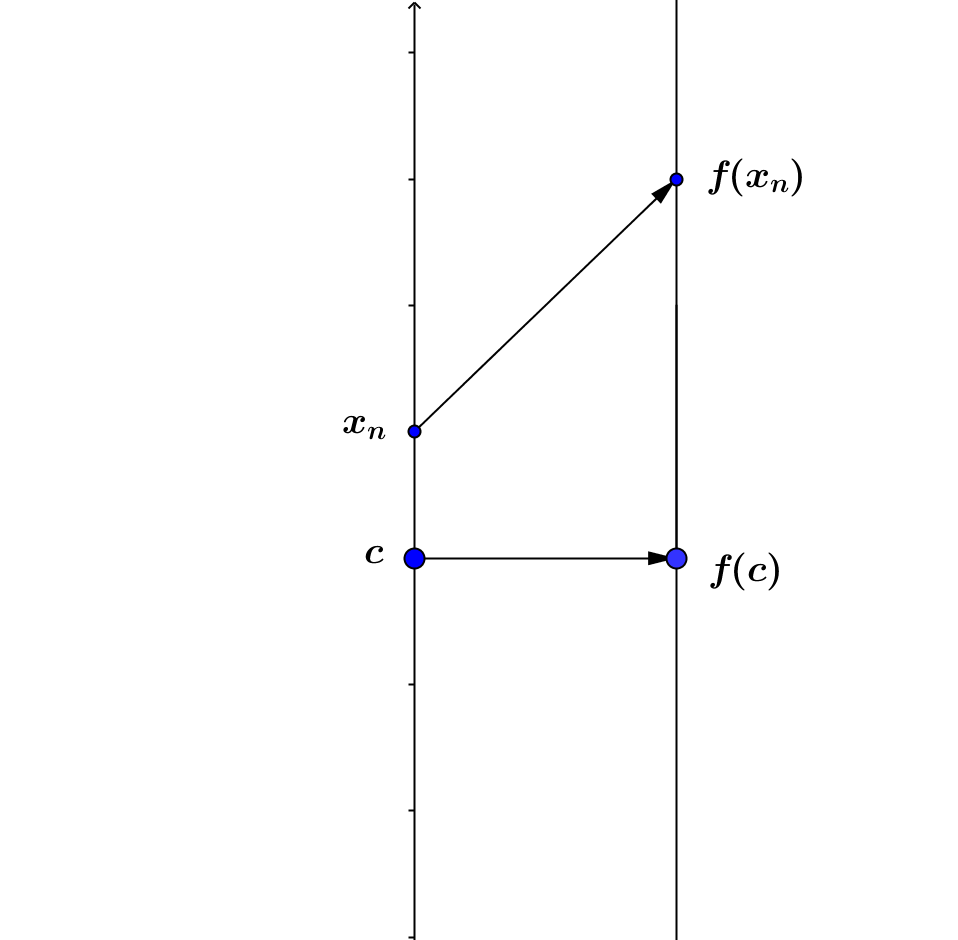Proof:
If f is not differentiable at c then the first alternative is satisfied and the theorem is true.

We may assume then that we are considering a situation where f(c) is an extreme value for f and f is differentiable at c.
Let's assume that f(c) is actually the minimum value of f and leave the case when it is the maximum value as an exercise.
Since f(c) is the minimum value for f(x), f(x) \ge f(c) for any x, so f(x) - f(c)\ge 0 for any x. Consider x_n > c and x_n \to c. [See Figure].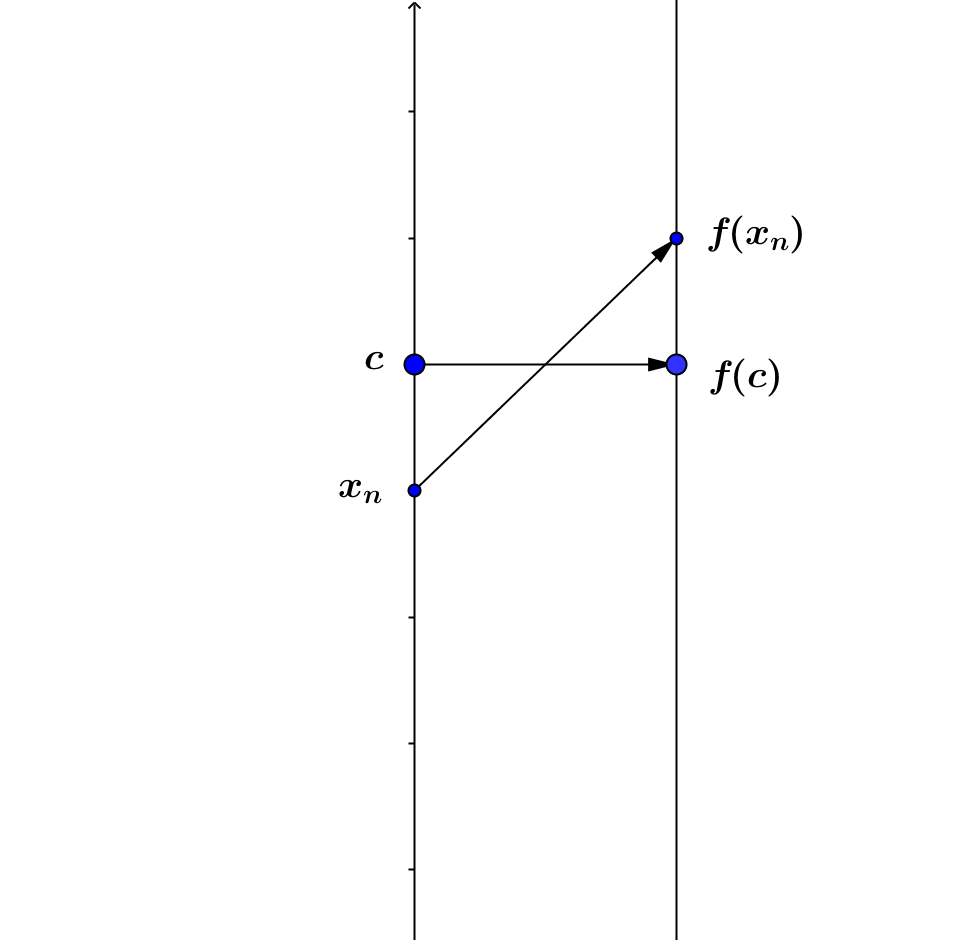In this case x_n - c > 0 so \frac{f(x_n) - f(c)} {x-c} \ge 0. But this means that f '(c) cannot be negative because there are quotients as close as we want to f '(c) that are non negative.
When we consider  x_n<c and x_n \to c then x_n - c<0 so \frac{f(x) - f(c)}{x-c} \le 0. But this means that f '(c) cannot be positive. [See Figure.]
So the only possibility for f '(c) is that f '(c) = 0, because we have assumed that f '(c) exists.
EOP.

3-4

The Flashman version of the brief history of integration... from Euclid, Archimedes, and Aristotle to Newton, Leibniz, Cauchy, and Euler.

Key concepts: Measurement of  curves and planar and spatial
regions. Estimation with finite approximations, use of proportions and trichotomy law, the "method of exhaustion". See Euclid Book XII  Proposition 2 Circles are to one another as the squares on their diameters.
Archimedes physical balancing with infinitesmals or indivisibles.    The Method of Archimedes
The Lost Book of Archimedes : Documentary on the Lost Manuscript of the Mathematical Genius [youtube (48:22)]

Introduction of decimals, logarithms, Descartes "analytic geometry" allows interpretation of higher powers of numbers as lengths, general solution of the area problems for most algebraic curves - except hyperbolae.

Galileo connects position and motion to areas in solving the problem of motion with constant acceleration. The development of logarithms as tools for solving trigonometric proportions and the creation of the hyperbolic/natural logarithm from an area problem.

Barrow's theorem relates area and tangent problems.
Newton considers curves as related to motion and velocity determines "slope of tangent line".
Leibniz uses infinitesmals to find area of regions determined by variables that related as functions.

Inability to find simple arithmetic formulae to determine "integrals" leads to desire for more precision in estimation and more accurate definitions returning to precision of Euclid and the method of Exhaustion to justify and clarify the key concepts used in the calculus of
Euler and Cauchy.

For alternative views see
Vignettes of Ancient Mathematics by Henry Mendell, Cal. State U., L.A. Special Topics
http://en.wikipedia.org/wiki/Mathematical_analysis#History.
and http://en.wikipedia.org/wiki/Timeline_of_calculus_and_mathematical_analysis

The Mechanical Universe -Ep7- Integration - YouTube

3-5 Beginnings of Integration: An interval [a,b] with a < b.
A partition of the interval: P = {a=x_0 < x_1 < x_2< ... < x_{n-1} < x_n=b}.
The partition P determines closed intervals [x_k, x_{k+1}] where k = 0, 1, 2, ... , n-1.
These intervals give rise to numbers:Deltax_k = x_k - x_{k-1} for k = 1,2,3,..., n.

Note: Euler used a partition where Deltax_j =Deltax_k  for all j and k , giving
Sigma_{k=1}^nDeltax_k = Sigma_{k=1}^nDeltax_1 = nDeltax_1 = b - a. So, for Euler Deltax = {b-a}/n.

Continuation: How to define the definite integral:

Suppose f is a function, f: [a,b] -> R. We can interpret f as the height of a curve above the "x" axis or as the velocity of an object moving on a straight line at time x.
We can define various sums based on an Euler partition of the interval with n equally sized sub-intervals:
L_n : Sigma_{k=1}^{n} f(x_{k-1}) Delta x [A Left Hand Sum]
R_n : Sigma_{k=1}^{n} f(x_k) Delta x [A Right Hand Sum]
If we let m_k = {x_{k-1} + x_k}/2 for k = 1,2,... ,n then
M_n : Sigma_{k=0}^{n-1} f(m_k) Delta x [A Midpoint Sum].

With these Euler sums we have three sequences determined by the function f and the interval [a,b].
First provisional attempt to define an integral based on experience using these sums in Calculus numerical integrals:
If there is a number I where  lim_{n->oo}  L_n = lim_{n->oo}  R_n  =lim_{n->oo}  M_n = I then we say that f is integrable over [a,b] and we denote I = \int_a^b f.
Comment: In calculus courses it is usually asserted that these approximations all work for continuous function, as well as other related estimates.
T_n = {L_n + R_n}/2  Trapezoidal estimate.
S_n = 2/3 M_n + 1/3 T_n  Simpson's (Parabolic) estimate.
It is not hard to see also that lim_{n->oo}  T_n = lim_{n->oo}  S_n   = I

If we try to use this definition we would want to basic properties to hold true:
1. If f(x) >= 0 for all  x in [a,b] then \int_a^b f >= 0.
2. If c in (a,b), then  \int_a^b f =  \int_a^c f  + \int_c^b f .

Example: Consider  f(x) = 1 if  x is rational and f(x) = 0 if  x is irrational.
On [0,1] we have L_n=R_n=M_n = 1 for all n, so \int_0^1 f = 1.
However on [1, sqrt{3}]  we have L_n= 1/n; R_n=M_n = 0 for all n, so \int_1^sqrt{3} f = 0.
And on [0, sqrt{3}]  we have L_n= {sqrt{3}}/n; R_n=M_n = 0 for all n, so \int_0^sqrt{3} f = 0.
But then if this definition of integration also satisfies property 2 we have
\0 = int_0^sqrt{3} f =  \int_0^1 f  + \int_1^sqrt{3} f  = 1+0=1.
If property 2 is going to hold then this function  leads to a contradiction.  This is an example of why we need to be more careful in defining the definite integral.

3-8
General Euler Integral:  Choose a set C with n points C = {c_1, c_2, ... , c_n} where c_k in [x_{k-1},x_k] [An Euler set].
Then define S_C = Sigma_{k=1}^{n} f(c_k) Delta x The general Euler sum that depends on C.
We  say that  f  is Euler integrable over [a,b] if there is a number I so that as n -> oo, the sums S_C -> I. If I exists it is called the Euler integral of f over [a,b] .
Since the size of the intervals Delta x = {b-a}/n -> 0 we can make this more precise:
We say lim_{n->oo}  S_C = I if given any epsilon >0, there is a natural number M so that if n > M and C is an Euler set with n points, then |S_C - I | < epsilon.

Example revisited: Consider  f(x) = 1 if  x is rational and f(x) = 0 if  x is irrational.
On the interval [0,1]  for any n use c_k = x_k. Then for any n, S_C =1.
But for any n, choose r_k in [x_{k-1},x_k]  where r_k is irrational. Then for C = {r_1,r_2, ..., r_n} we have S_C = 0.
Thus the function f is not "Euler" Integrable.

Discussion of integration and connection to continuity.
3-10&11
• Various definitions and an example of a function that is not integrable.

Week 10
3-21 through 3- 25
These lectures and notes are found on a separate link: Integration Notes from Week 10
Discussion of assignment due March 8 problem 5:
Suppose f is continuous  on [a,b] and for all x,y in [a,b] if x<y then f(x) <f(y) .
a. f([a,b]) = [f(a),f(b)].
b. There is a unique function g: [f(a),f(b)] -> [a,b] where g(f(t)) = t for all t in [a,b] and f(g(s)) = s  for all s in [f(a),f(b)].
c. g is continuous.
Part a - Use definitions and assumptions to prove the set equality. [IVT used.]
Part b. Show f is one to one.... then review of  "inverse functions" from Math 240.
Proof of c.
Consider a sequence {c_n} where lim c_n = c in [f(a),f(b)]. Then let r_n = g(c_n) in [a,b]  so f(r_n) = c_n. Since {r_n} is a bounded sequence, using BW Theorem we have a convergent subsequence {s_m} where s_m = r_n for some n>m and lim s_m = s. Thus f(s_m) = f(r_n) =c_n and by continuity of f, lim f(s_m) = f(s) = lim c_n = c. Thus g(c)= s. It suffices to show that lim r_n = s.
Suppose not. Then there is an epsilon >0 where for any natural number M there is a number j > M where |r_j - s| > epsilon .   Then for all j,  s>z = s- epsilon > r_j  or s< w = s+epsilon< r_j. Thus c= f(s) > f(z) > f(r_j)=c_j  or c = f(s)< f(w)< f(r_j) = c_j. But this contradicts lim c_n = c. Thus lim  r_n = lim g(c_n) = s = g(c) and g is continuous.

• FTofC and inverse function theorem connected to Ln and e^X , Arctan and tangent functions, Arcsin and sin functions can be defined through integration and Inverse Function Theorem.
• Bounded Function Constraint Result for Integral.

Main properties of the definite integral that we will prove:
Including: Monotonicity. Linearity. Additivity. Bounded Constraint. Continuity of Integral Function for integrable functions. Continuous Functions are integrable. Fundamental Theorem (Derivative form) for Continuous Functions. FTofC (Evaluation form) for Continuous Functions. Mean Value Theorem for Integrals for Continuous Functions.

Additivity. Continuity of Integral Function for Integrable Functions. Continuous Functions are Integrable.
FTof Calc I and II, MVT for Integrals. Alternative proof of FTofC using MVT for Integrals.
Discussion of Improper Integral for Discontinuities on bounded intervals. Sets of Measure Zero introduced. Countable Sets have measure zero. Continuity, integrability, and measure zero sets related. The Cantor Set: has measure 0 but is uncountable.

Week 11
3-28

Non-sequence based limits and continuity.

Limits of sequences with intervals:
Note: lim_{n ->oo} a_n = a if for any epsilon >0 there is a number M in N where if n>M then |a_n -a| < epsilon.
Rephrase:..... a_n in ( a-epsilon, a+epsilon).

Definition of an open set.
Suppose O  is a set of real numbers. O is open if (and only if) for any a in O there is a epsilon > 0 where ( a-epsilon, a+epsilon)  sub  O.

Example: The interval (1,3) is an open set.
Proof: Choose  a in (1,3). Let  epsilon = 1/2 min{a-1, 3-a}. Suppose x in ( a-epsilon, a+epsilon).  Then 1= a-(a-1)\le a- min{a-1,3-a}< a-epsilon< x < a+epsilon < a + min{a-1,3-a} \le a+ (3-a)=3. Thus x in (1,3) , ( a-epsilon, a+epsilon)  sub (1,3)  and (1,3) is open. EOP

Example: The interval (1,3] is not an open set.
Proof:  Consider  a=3 in (1,3]. Choose any epsilon >0. Then 3 +epsilon/2 in  ( a-epsilon, a+epsilon) but 3 +epsilon/2 is not an element of (1,3] so for any epsilon >0, ( 3-epsilon, 3+epsilon) is not a subset of (1,3]  and thus (1,3] is not an open set. EOP

Remark: It can be proven similarly that for any a < b ,  (a,b)  is an open set.

Note continued:
lim_{n ->oo} a_n = a: if for any open set,O, with a in O, there is a number M in N where if n>M then a_n in O.

Continuity: With sequences:   f  is continuous on its domain D if for any a in D and  for any sequence a_n in the domain of f where lim_{n->oo}a_n = a,  lim_{n->oo} f(a_n) = f(a).
With sequences and open sets:  If  a_n
is a sequence in the domain of f where lim_{n->oo}a_n = a then for any open set O with f(a) in O, there is an number M in N where if n>M then f(a_n) in O.

epsilon -delta   Definition of Limits and Continuity.
The limit of f  as x -> a for a in D is L if for any epsilon >0, there is a real number delta>0 where if x in D and 0<|x-a|< delta , then |f(x)-L|<epsilon. This is written as lim_{x ->a} f(x) = L.
f  is continuous on its domain D if for any a in D and epsilon >0, there is a real number delta>0 where if x in D and |x-a|< delta , then |f(x)-f(a)|<epsilon. That is, for any  a in D , lim_{x ->a} f(x) = f(a).

See these on-line references:
GeoGebra Mapping Diagram for limits: http://ggbtu.be/mNLaWHLas
Continuity of Real functions
Images of intervals

Limits of functions

3-29

Example: Let f(x) = C, a constant function. Then f is continuous for the set of real numbers.
The justification of this statement is left as an exercise for the reader.

Example: Let f(x) = 3x+5, a linear function. Then f is continuous for the set of real numbers.
Proof:  Suppose a in R and epsilon > 0.
[ We worked with inequalities to see that a likely effective choice for delta was epsilon /3.]
Let delta = epsilon/3. Then suppose x in R and  |x-a| < delta = epsilon/3.
Then |f(x)-f(a)| = |(3x+5) - (3a+5) | = |3(x-a)| = 3|x-a|.
But we assumed |x-a| < epsilon/3 so |f(x)-f(a)| = 3|x-a| < 3 epsilon/3 = epsilon.
Thus f is continuous for every real number.   EOP

For a visualization of epsilon-delta proof of continuity for linear functions see this GeoGebra worksheet:

http://ggbtu.be/mfvWrWyBu

Compare the previous proof with the sequence based proof:
Suppose a_n and lim_{n->oo} a_n =a. Then by applying the results we have about limits of sequences,lim_{n->oo} f(a_n) = lim_{n->oo} 3a_n+ 5 = 3a +5 = f(a).

Theorem: Suppose f is a function with domain D.
f is  epsilon-delta continuous on D if and only if f is sequence continuous on D.
Proof:
(1) "only if" :  Suppose f is epsilon-delta continuous on D. Assume a in D and a_n in D with   lim_{n->oo} a_n =a. Given epsilon > 0, there is a delta where if x in D and |x-a| < delta then |f(x) - f(a)| < epsilon. Since  lim_{n->oo} a_n =a there is a number M in N where if n > M then |a_n -a| < delta and hence |f(a_n)-f(a)| < epsilon. Thus  lim_{n->oo} f(a_n) = f(a) and f  is sequence continuous on D.

(2) "if" :  Assume f is sequence continuous on D. This will be indirect - so we assume f is not epsilon-delta continuous on D.  Then there is an element of D, a* and a positive real number, epsilon* > 0 where for any delta > 0 there is an x in D with |x-a*| < delta while |f(x) -f(a*)| \ge epsilon*.

Now use delta = 1/2 and let x_1 in D be so that  |x_1-a*|< 1/2 while |f(x_1) -f(a*)| \ge epsilon*.
Next  use delta = 1/4 and let x_2 in D be so that  |x_2-a*|<1/4 while |f(x_2) -f(a*)| \ge epsilon*.
Continuing  use delta = 1/2^n and let x_n in D be so that  |x_n-a*|<1/2^n while |f(x_n) -f(a*)| \ge epsilon*.

The sequence x_n in D has  lim_{n->oo} x_n =a* but lim_{n->oo} f(x_n) ne f(a*). This contradicts the assumption that f  is sequence continuous on D.  EOP

See the following link for a similar presentation of the argument.
The epsilon-delta definition : Equivalence of definition with sequence definition.

4-1 and 4-4
Open, closed, bounded. Openness and continuity.
Open and closed sets in metric spaces (consider the  real numbers with the metric given by the absolute value of the difference).[Topology]

Notation: N(L, epsilon) = ( L - epsilon, L+ epsilon) = {x: |x-L| < epsilon}.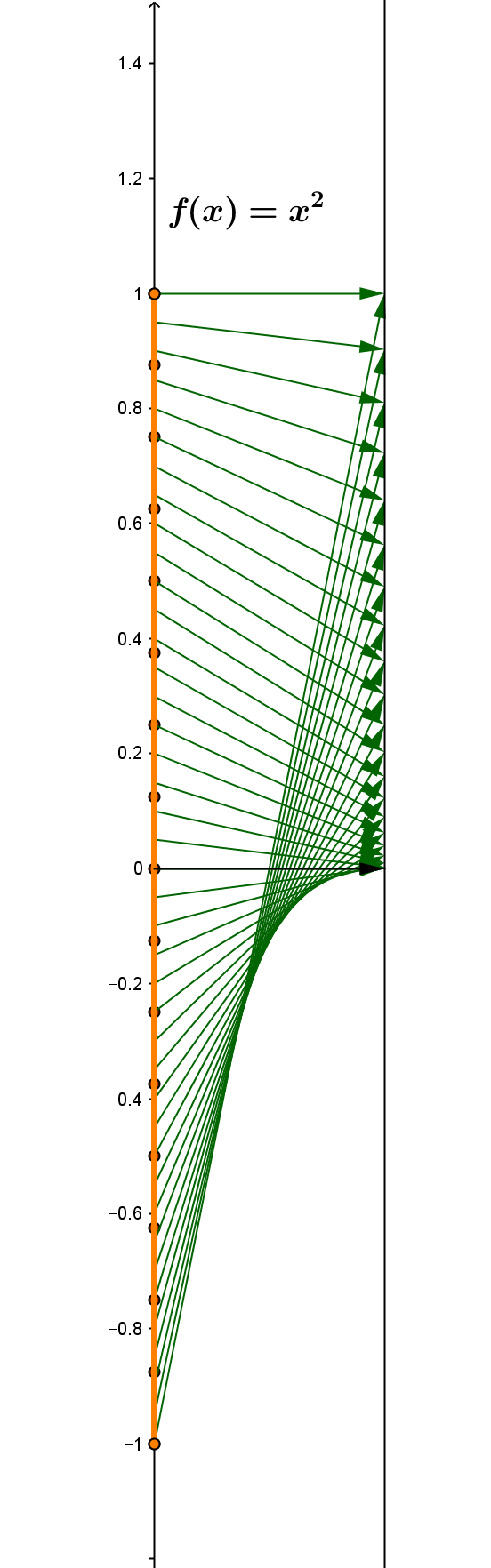Recall: Definition of an open set. O is open if for any L in O there is a epsilon > 0 where N(L, epsilon )sub O.

Review Definitions: Given f : D -> R, and A sub D

The image of A under f , f (A) =  { y in R :  y = f (x) for some x in A}and
the preimage of A under f,  f ^{-1}(A) = {x in R : f (x) in A}.
Example: f(x) = x^2.
f ^{-1} $$\[0\,1$$\)= (-1,1) ;
f ^{-1} $$\(0\,1$$\)= (-1,0) cup (0,1);
f ^{-1} $$\(1,4$$\)= (1,2) cup (-2,-1).

Continuity and open sets

4-5
Theorem: Given
f : R ->R , f  is epsilon- delta continuous if and only if  whenever O is an open subset of R, f ^{-1}(O) is also an open set.
["A function is continuous if and only if the inverse image of an open set is open."]

Proof:  (1)  => :  Suppose f is epsilon-delta continuous on D.
Suppose O is an open set and that a in f ^{-1}(O). Then f(a) in O. Since O is an open set, there is an epsilon >0 where N(f(a),epsilon) sub O. Since f is epsilon- delta continuous, there is a delta >0 where if x in N(a,delta) then f(x) in N(a,epsilon). Thus N(a,delta) sub f ^{-1}(O) and f ^{-1}(O) is an open set.

(2) <= :  Suppose that whenever O is an open subset of R, f ^{-1}(O) is also an open set.
Suppose epsilon >0 and a in R. Then N(f(a),epsilon) is an open set with a in f ^{-1}(N(f(a),epsilon)). By the hypothesis f ^{-1}(N(f(a),epsilon)) is an open set, so there is a number delta>0 where N(a,delta) sub f ^{-1}(N(f(a),epsilon)).  Then if x in N(a,delta) then f(x) in N(a,epsilon) and f is epsilon-delta continuous.
EOP.

Prop: (i) O/ and R are open sets.
(ii) If U and V are open sets,  then U nn V is an open set.
(iii) If   O is a family of open sets, then uuu O = { x in R : x in U for some  U in  O} is an open set.

4-7
Proof of (ii)
Suppose a in U nn V. Then  a in U and a in V.  Since U is an open set, there are numbers epsilon_U where N(a, epsilon_U) sub U and  epsilon_V where N(a, epsilon_V) sub V. Let epsilon = min(epsilon_U, epsilon_V). Then it is not hard to show that N(a,epsilon) sub U nn V and thus U nn V is an open set. EOP

Proof of (iii)
Suppose a in uuu O. Then  a in U for some U in O.  Since U is an open set, there is a number epsilon_U where N(a, epsilon_U) sub U. Let epsilon = epsilon_U  Then it is not hard to show that N(a,epsilon) sub uuuO and thus uuu O is an open set.
EOP

Fact:If  a in R then (a, oo) and (-oo, a) are open sets.
Fact: {a} is not an open set.

Cor. : If a < b , then (a,b) is an open set.

The family of all open sets of R is sometimes called the topology of R.
Example: Let U_n = (-1/n,1/n). Then uuu{U_n} = (-1,1) which is open. BUT nnn{U_n} = { 0} which is not open.

Continuity and open sets

Sidenotes:
A topological space is a set X together with a family of subsets O that satisfies the three properties:
(i) O/ in O and X in O.
(ii) If  U , V in O ,  then U nn V in O.[Closure under "finite intersection".]
(iii) If   {U_{alpha}} is a family of sets with each U_{alpha} in O, then uuu U_{alpha} = {x in X : x in U_{alpha} for some  U_{alpha} in the family}in O. [Closure under "arbitrary union".]

Definition of a closed set. A set C is closed if (and only if) R - C is open.
Prop: (i) O/ and R are closed sets.
(ii)
If  a in R then [a, oo ) and (- oo, a] are closed sets.
Fact: {a} is a closed set. [ R-{a} = (-oo,a)uu(a,oo) which is an open set.]
4-8
Prop: (i) If U and V are closed sets,  then U uu V is a closed set.
(ii) If   K is a family of closed sets, then nnn K = { x in R : x in C for all  C in  K} is a closed set.
Cor. : If a < b , then [a,b] is a closed set.
Proof: [a,b]= [a,oo) nn (-oo,b].

Connectedness , Continuity, and Topological Proof of the Intermediate Value Theorem.

Def'n: A subset I of the R is an interval: If a, b in I  and a < x< b, then xinI.
Def'n: A subset S of (a metric space) R is disconnected if there are open sets U and V where
(i) U uu V sup S; (ii)
U nn S != O/ , V nn S != O/ , AND V nn U = O/.
Def'n: A subset S of (a metric space) R is connected if it is not disconnected.
Thus, if S is connected with
open sets U and V where
(i) U uu V sup S;
and (ii) U nn S != O/ , V nn S != O/, then V nn U != O/.

The following result leads immediately to the IVT.
Theorem:
A subset S of R is connected if and only if it is an interval. [Skip to IVT]
Proof: =>:
[Indirect- contrapositive] Suppose S sub R and S is not an interval. Then there exist a in S and b in S with a<b and c where a<c<b and c not in S. Let U = (-oo,c) and V=(c,oo). Then U uu V sup S; a in U nn S  so U cap C!= O/ and b in V nn S  so V cap C!= O/ and certainly V nn U =O/, so S is not connected.

<=:  Deferred till 4-11
Suppose S is an interval. To show S is connected, suppose open sets U and V where
(i) U uu V sup S; and (ii) U nn S != O/ , V nn S != O/ .
[We need to show that V nn U != O/.] Also suppose V cap U = O/.
Choose a in U cap S and b in V cap S. For convenience assume a<b.
Let R = {t : [a,t] sub U}.

Since  a in R, R !=O/. Since b in V , if x in R then x < b and thus R is nonempty and bounded above, so by the least upper bound property of the real numbers we can
let z = lub(R).
Then a le z le b and since S  is an interval, z in S. So either z in U or z in V. [We will show that neither of these alternatives are possible.]

(a) Suppose z in U.
Since U is open, there is an epsilon_U>0 where N(z,epsilon_U) sub U. Then z-epsilon_U <z is not an upper bound for R and there is w in R where z-epsilon_U < w le z. But then [a,w]sub U and [a,z+{epsilon_U}/2] = [a,w]cup [w,z+{epsilon_U}/2] sub U. Thus z+{epsilon_U}/2 in R  and z is not an upper bound for R. So z!in U.

(b) Suppose z in V.
Since V is open, there is an epsilon_V>0 where N(z,epsilon_V) sub V. Then z-epsilon_V <z is not an upper bound for R and there is w in R where z-epsilon_V < w le z. But then [a,w]sub U. But then w in U while w in N(z,epsilon_V) so w in V. This contradicts U cap V=O/ so  z!in V.

EOP.

Theorem: If f : R -> R  is continuous and C is a connected set, the f(C) is a connected set.
Proof: Suppose not. Then let U and V  be open sets and
(i) f(C) sub U uu V ; and (ii)  U nn f(C) != O/ , V nn f(C) != O/, AND V nnU = O/ . We will show this implies that R is not a connected set.
Since f is continuous for R,  hat U =f ^{-1}(U) and hatV =f ^{-1}(V) are open sets.
Now (i) hatU uu hatV sup R ; and (ii) hatU nn R!= O/ , hatV nn R != O/ , AND hatU nn hatV = O/. [why?]
But this contradicts the fact that R is a connected set. EOP.

More on Connected and disconnected sets

IVT.
Theorem: If f : R -> R  is continuous and a<b and v is between f(a) and f(b) then there is a number c in [a,b] so that f(c) = v.

Proof:
Suppose not. Then let U= (-oo,v) and V= (v,oo) and
(i) f([a,b]) sub U uu V ; and (ii)  U nn f([a,b]) != O/ , V nn f([a,b]) != O/, AND V nnU = O/ .  So f([a,b] is not connected. but [a,b] is a connected set, so f([a,b]) is connected.

Cor: If
a<b and f : [a,b]-> R is continuous and v is between f(a) and f(b) then there is a number c in [a,b] so that f(c) = v.
Proof:
Extend the function f by letting f(x) = f(a) for x < a and f(x) =f(b) for x>b. Then f: R->R and f is continuous so the previous theorem can be applied. EOP.

4-11 Deferred from  4-8
Theorem: A subset S of R is connected if and only if it is an interval.
Proof <=:Suppose S is an interval. To show S is connected, suppose open sets U and V where
(i) U uu V sup S; and (ii) U nn S != O/ , V nn S != O/ . [We need to show that V nn U != O/.] Also suppose V cap U = O/.
Choose a in U cap S and b in V cap S. For convenience assume a<b.
Let R = {t : [a,t] sub U}.
Since  a in R, R !=O/. Since b in V , if x in R then x < b and thus R is nonempty and bounded above, so by the least upper bound property of the real numbers we can let z = lub(R).
Then a le z le b and since S  is an interval, z in S. So either z in U or z in V. [We will show that neither of these alternatives are possible.]

(a) Suppose z in U.
Since U is open, there is an epsilon_U>0 where N(z,epsilon_U) sub U. Then z-epsilon_U <z is not an upper bound for R and there is w in R where z-epsilon_U < w le z. But then [a,w]sub U and [a,z+{epsilon_U}/2] = [a,w]cup [w,z+{epsilon_U}/2] sub U. Thus z+{epsilon_U}/2 in R  and z is not an upper bound for R. So z!in U.

(b) Suppose z in V.
Since V is open, there is an epsilon_V>0 where N(z,epsilon_V) sub V. Then z-epsilon_V <z is not an upper bound for R and there is w in R where z-epsilon_V < w le z. But then [a,w]sub U. But then w in U while w in N(z,epsilon_V) so w in V. This contradicts U cap V=O/ so  z!in V.

Continuous functions and connected sets

A topological analysis for the Extreme Value Theorem:

Compact sets(topological definition)
• Definitions: Suppose $F$ is a family of sets and $A⊂R$. We say $F$ is a cover of $A$ if uuuF={x:xU for some $U∈F}⊃A$.
If $F$ is a cover of $A$ and every $U∈F$ is an open set, then we say $F$ is an open cover of $A$.
If $F^$ is a subfamily of $F$, i.e., $F^⊂F$, and $F^$ is a cover of $A$, we say that $F^$ is a subcover of $F$.
• We say that a set $K$ is (topologically) compact if any open cover $F$ of $K$ has a subcover $F^$ that is a finite set, i.e., for any open cover $F$ of $K$, there exist $U1,U2,...,Un∈F$ where  $U1∪U2∪...∪Un⊃K$.
• Examples: i. A finite set is compact.
ii. ${1,12,13,...}={x:x=1n$ for $n>0,n∈N}$ is not compact. [This set is not closed!]
iii. ${0,1,12,13,...}={0}∪{x:x=1n$ for $n>0,n∈N}$ is compact.[This set is closed.]
iv. ${1,2,3,...}={x:x=n$ for $n>0,n∈N}$ is not compact. [This set is closed, but not bounded.]

•  Outline:
• Heine Borel Theorem (simplest version): $[a,b]$ is a compact subset of R.
• Theorem: A closed subset of a compact set is compact.
• Theorem: If $K$ is a compact subset of $R$, then $K$ is closed and bounded. [HB in R]
• Theorem: If  $f:R→R$ is continuous and $K$ is a compact  then  $f(K)$ is compact.
• Extreme Value Theorem (Topological Proof) Suppose f: [a,b] -> R is continuous. Then there existc_M and c_m in [a,b] where f(c_m) le f(x) le f(c_M) for all x in [a,b].
Proof: We can suppose that f(x) = f(b) for x > b and f(x) = f(a) for x<a, so f: R -> R is continuous.
Since [a,b] is compact, f([a,b]) is compact.
Since [a,b] is also an interval, f([a,b]) is a closed and bounded interval.
Thus f([a,b]) = [m,M] where for all x in [a,b], m le f(x) le M.
But since m, M in f([a,b]), there exist c_m and c_M in [a,b] where f(c_m) = m and f(c_M) = M.  EOP!

4-14 Proofs remaining from outline.

Heine Borel Theorem (simplest version): $[a,b]$ is a compact subset of R.

Proof:
(outline). Suppose O is a family of open sets where $uuuO⊃[a,b]$.
Let G= {x $∈$ $[a,b]$ where $[a,x]$ is covered by a finite subset of the members of O.}.
Claims: a in G because [a,a] = {a} and since [a,b] subset uuu O
,  for some U in O,  a in U.
Thus G
$≠$ $∅$ and by its definition G is bounded by $b$.
Then let $α$ = lub of G.
Plan: Show alpha = b and $α∈$G
alpha = b: Suppose alpha < b. Then a le alpha < b. Since O is an open covervof [a,b], there is an open set U_{alpha} where alpha in U_{alpha} in O and epsilon_{alpha} > 0 where N(alpha, epsilon_{alpha}) sub U_{alpha} nn [a,b].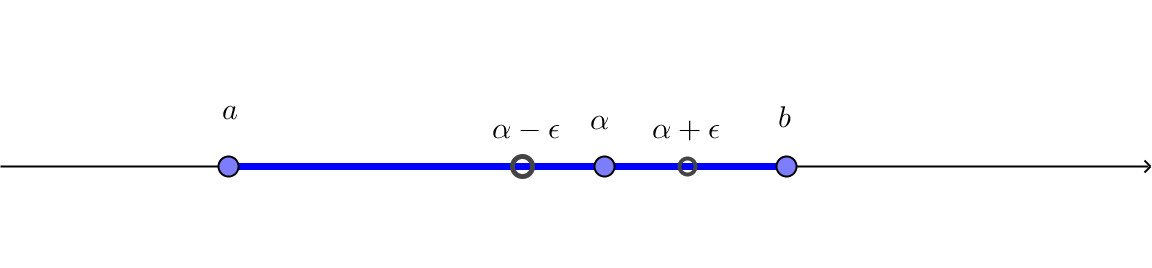Considering alpha - epsilon_{alpha} < alpha is not an upper bound for G  so there is a number c in G with alpha-epsilon_{alpha} < c<alpha.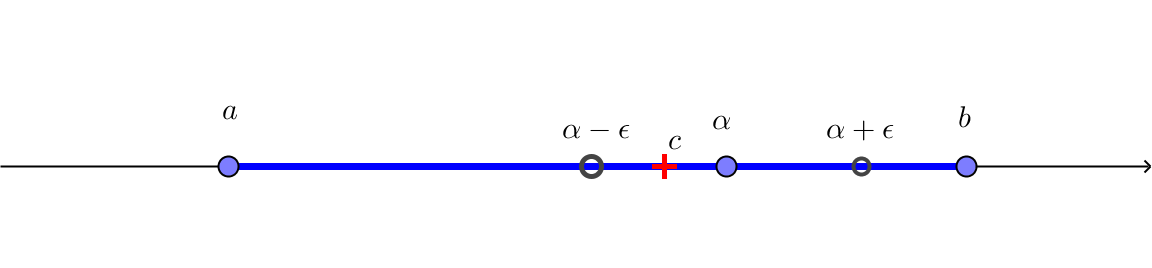Since c in G, the interval [a,c] is covered by a finite subset of the members of O.
But then add U_{alpha} to this family of members of O and we see that alpha + epsilon_{alpha}/2 < b is also in G which contradicts that alpha is an upper bound for G.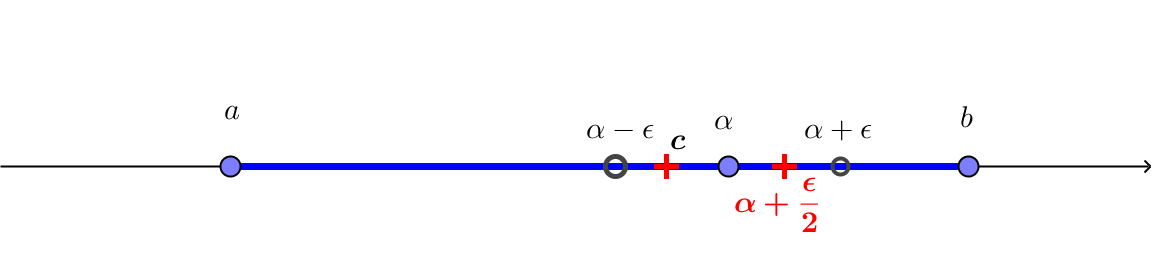So alpha =b.
α∈G: Using U_{alpha} from above, we have epsilon> 0 where  N(alpha, epsilon) sub U_{alpha}. Following an argument similar to the last one, alpha - epsilon < alpha is not an upper bound for G  so there is a number c in G with alpha-epsilon < c<alpha.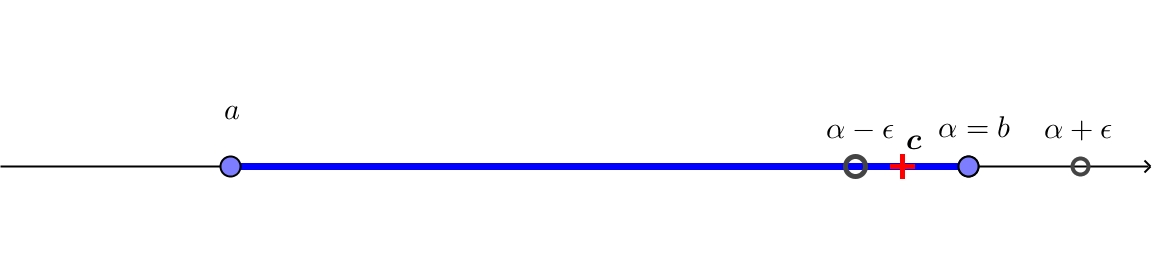Since c in G, the interval [a,c] is covered by a finite subset of the members of O.
But then add U_{alpha} to this family of members of O and we see that alpha = b is also in G.   Thus [a,b] is (topologically) compact.
EOP

• Theorem: A closed subset of a compact set is compact.
Proof:
Supposed $C$ is a closed set and $K$ is a compact set with $C⊂K$
Now suppose
O is a family of open sets where .
Since $C$ is closed, let U = R-C and $O^=O∪{U}$.
Then $O^$ covers $K$ and by compactness a finite subcover of $O^$ covers $K⊃C$.
But then if needed that finite subcover less $U$ will be a finite subcover of $O$ that covers $C$.
So $C$ is compact. EOP.

• Corollary: If K is a closed and bounded subset of R, then K is compact.
Proof: K is bounded- so K $⊂[m,M]$ for some $m$ and $M$. But by HB, $[a,b]$ is compact, so K is a closed subset of a compact set and thus K is compact. EOP

4-15
• Theorem: If $K$ is a (topologically) compact subset of $R$, then $K$ is closed and bounded. [HB in R]
Proof:
(i) $K$ is bounded:
Consider the family of open sets, $O={N(0,n)forn=1,2,3....}$. Certainly this family covers any subset of $R$, so it covers $K$. But a finite subcover will be bounded by $n+1$ for the largest $n$ of the sets in that finite subcover. Thus $K$ is bounded.

(ii) $K$ is closed.
Let $U$ =
R -K, the complement of  K. We will show that $U$ is open.
Suppose $p∈U$  and $x∈K$
Then let $r(x)=|x-p|3$ so $r(x)>0$